# RD Sharma Solutions for Class 6 Maths Chapter 9: Ratio, Proportion and Unitary Method

The solutions created by faculty at BYJU’S are very helpful for the students to score well in the exam. Gaining knowledge about the various concepts which are explained in Mathematics is very important as few topics are continued in higher classes as well. The solutions are mainly created with the aim of helping the students improve their problem solving and logical thinking abilities.

Students by downloading PDF of solutions obtain clear idea about the concepts covered in each exercise. The solutions contain explanations in an interactive manner to make the subject interesting for the students. RD Sharma Solutions for Class 6 Maths Chapter 9 Ratio, Proportion and Unitary Method PDF are provided here.

## RD Sharma Solutions for Class 6 Maths Chapter 9: Ratio, Proportion and Unitary Method Download PDF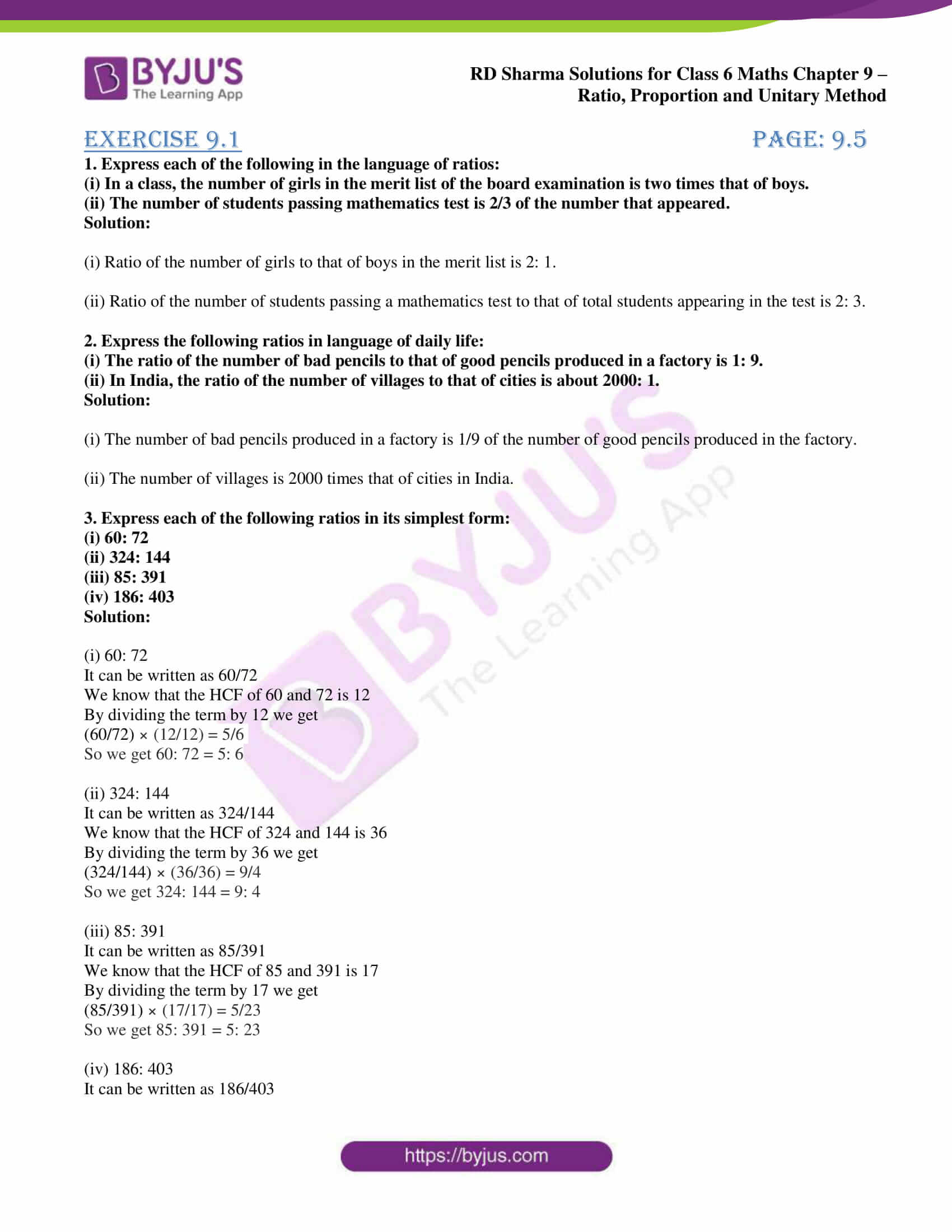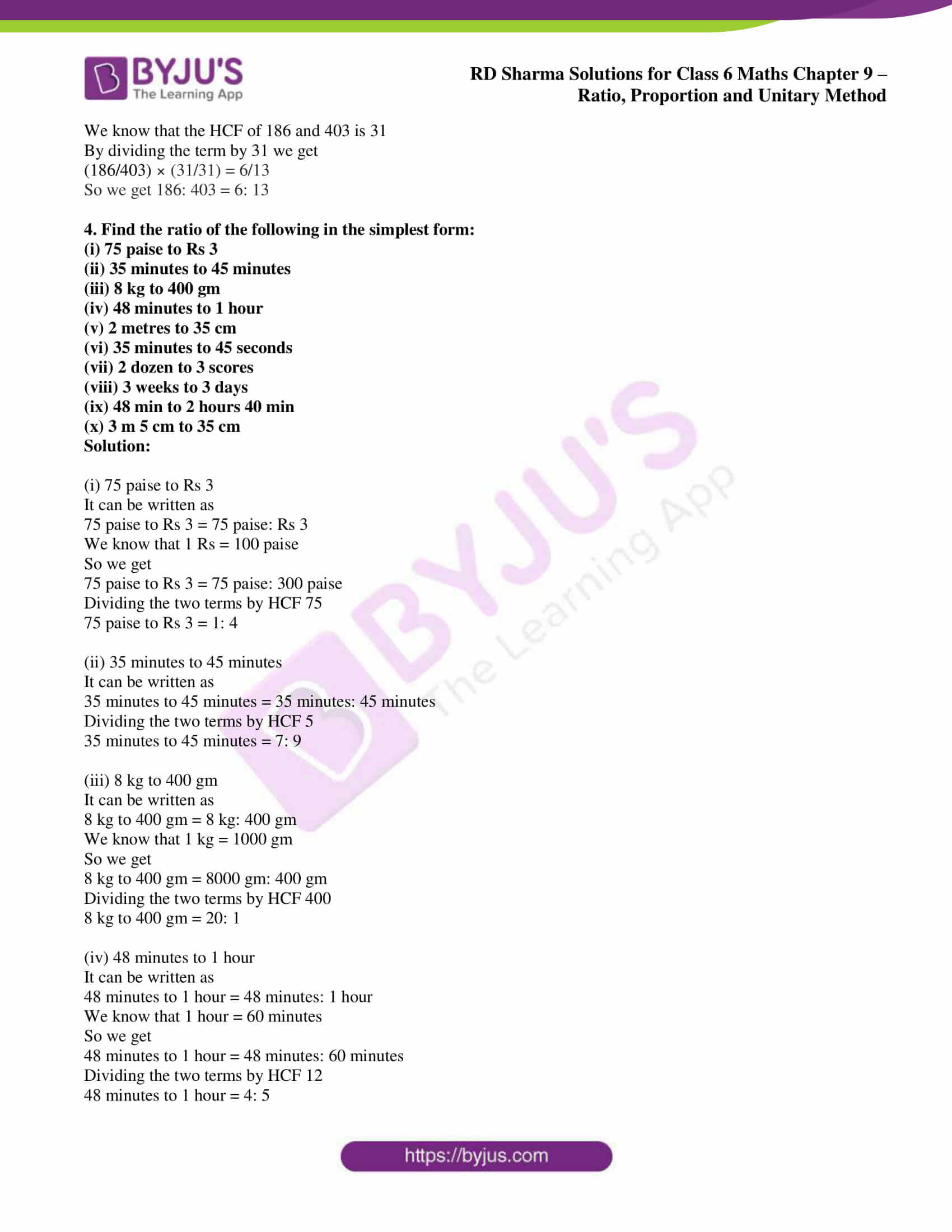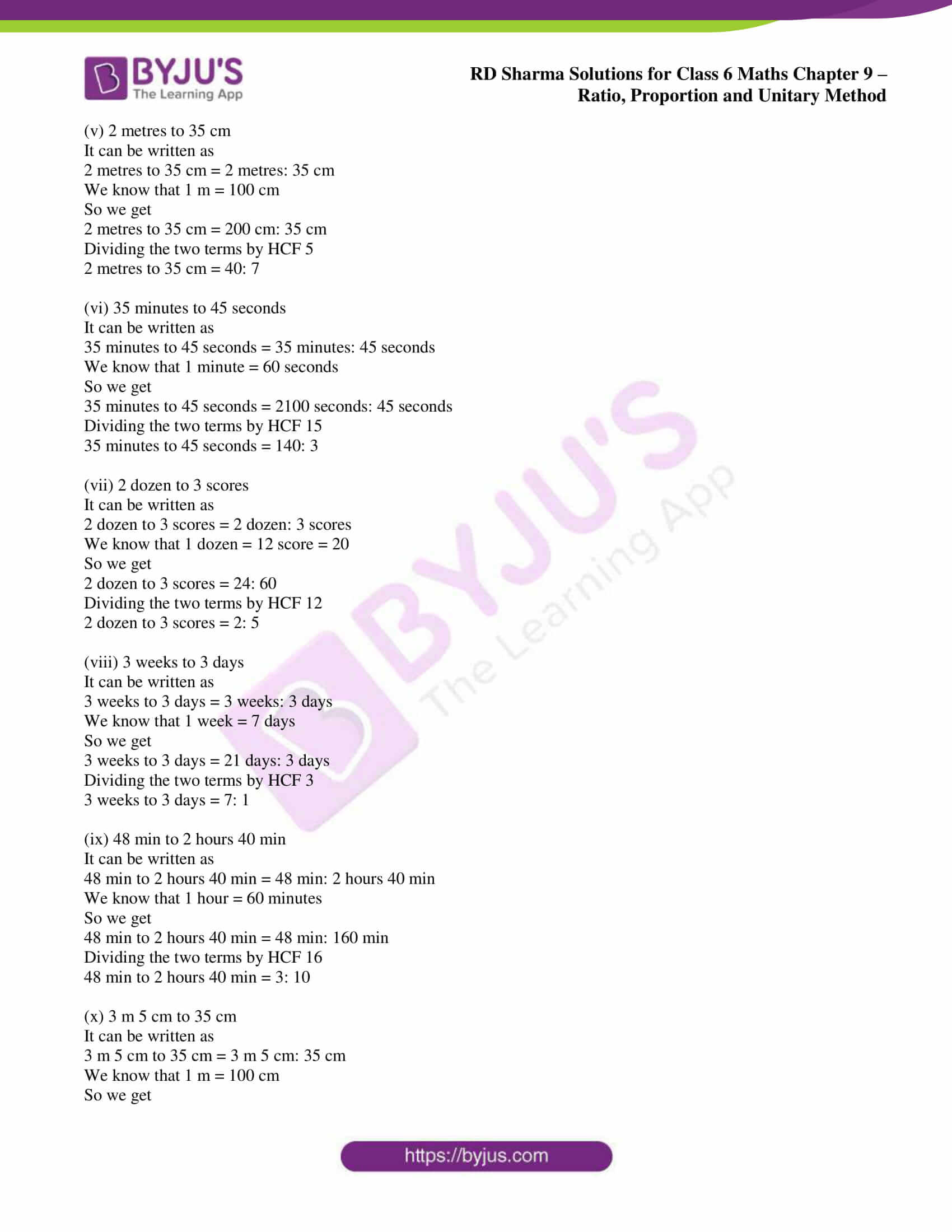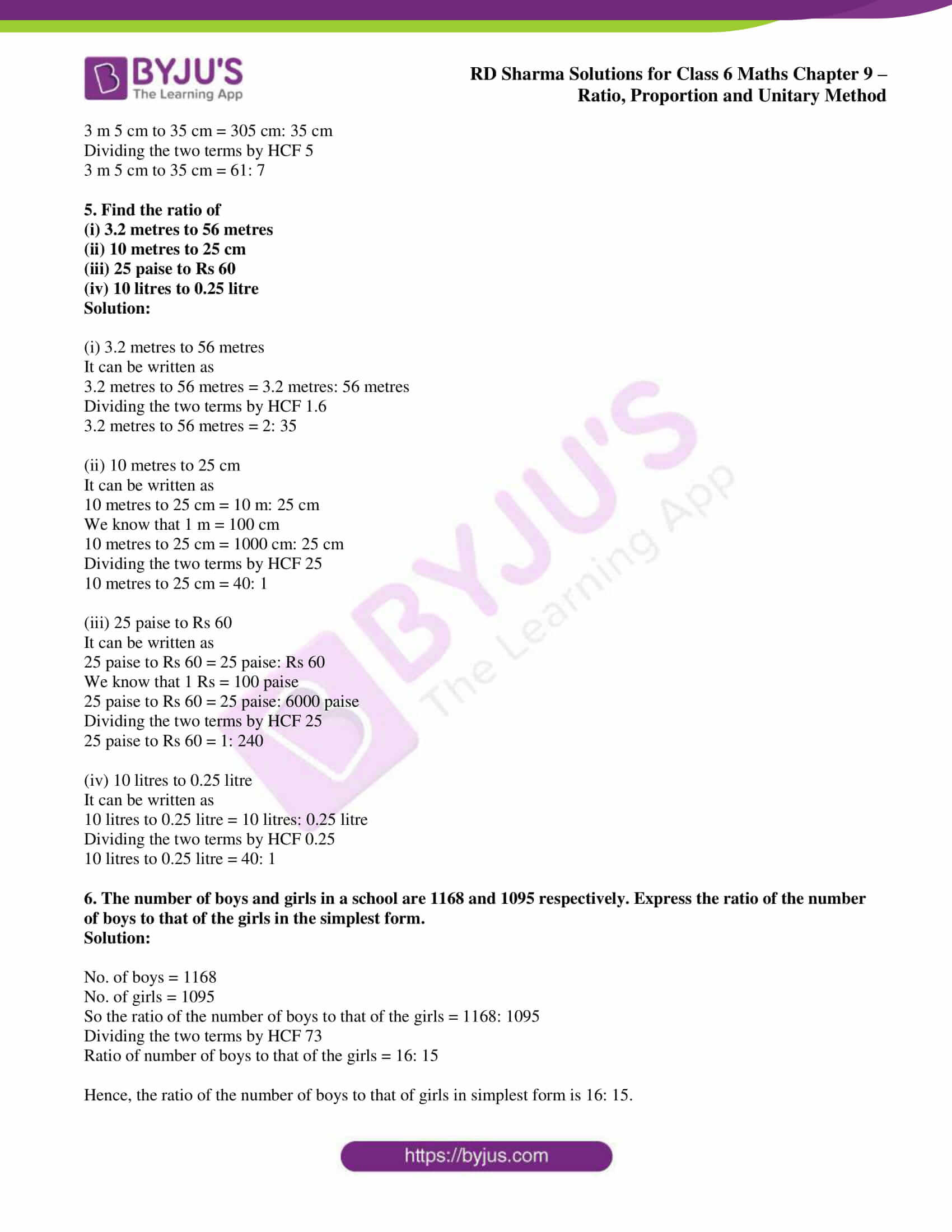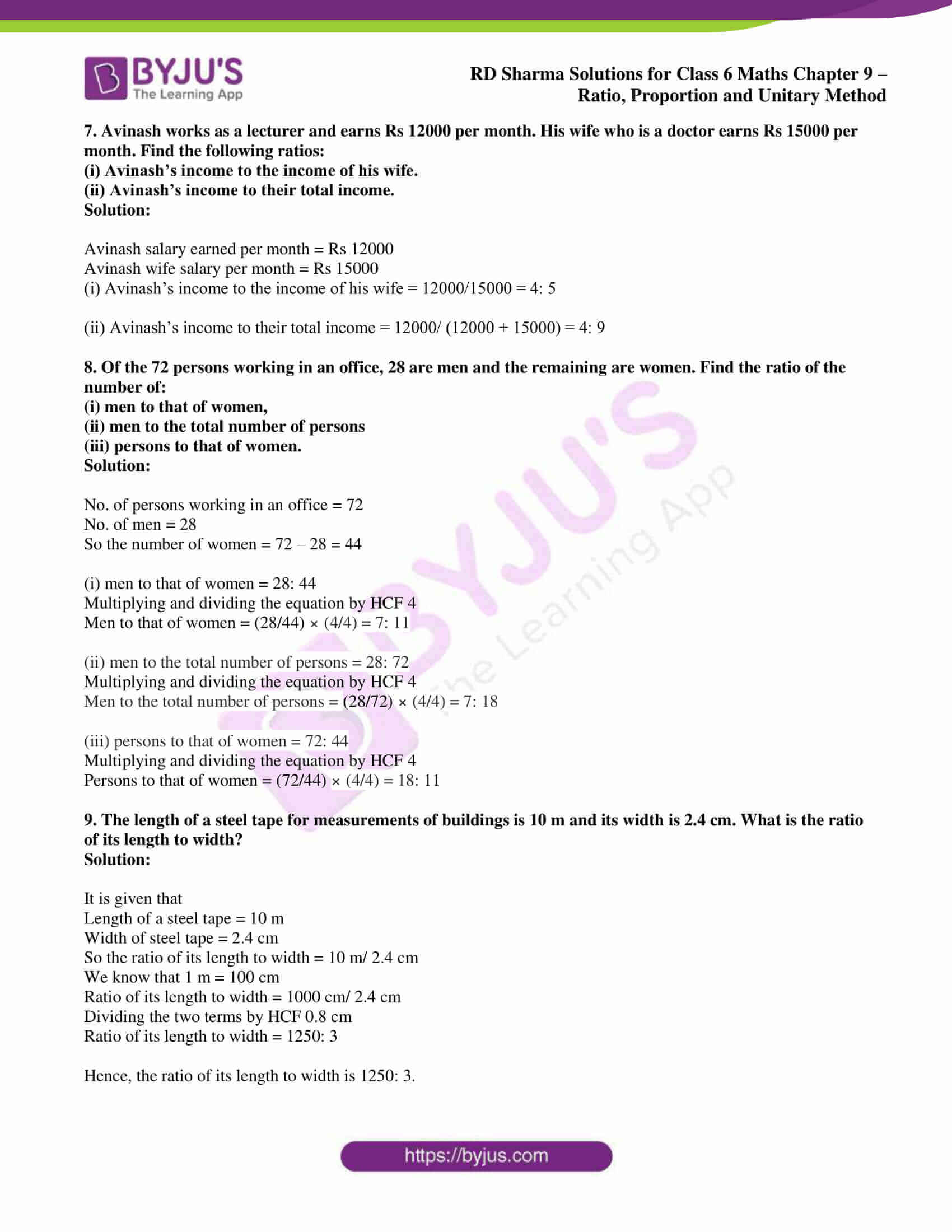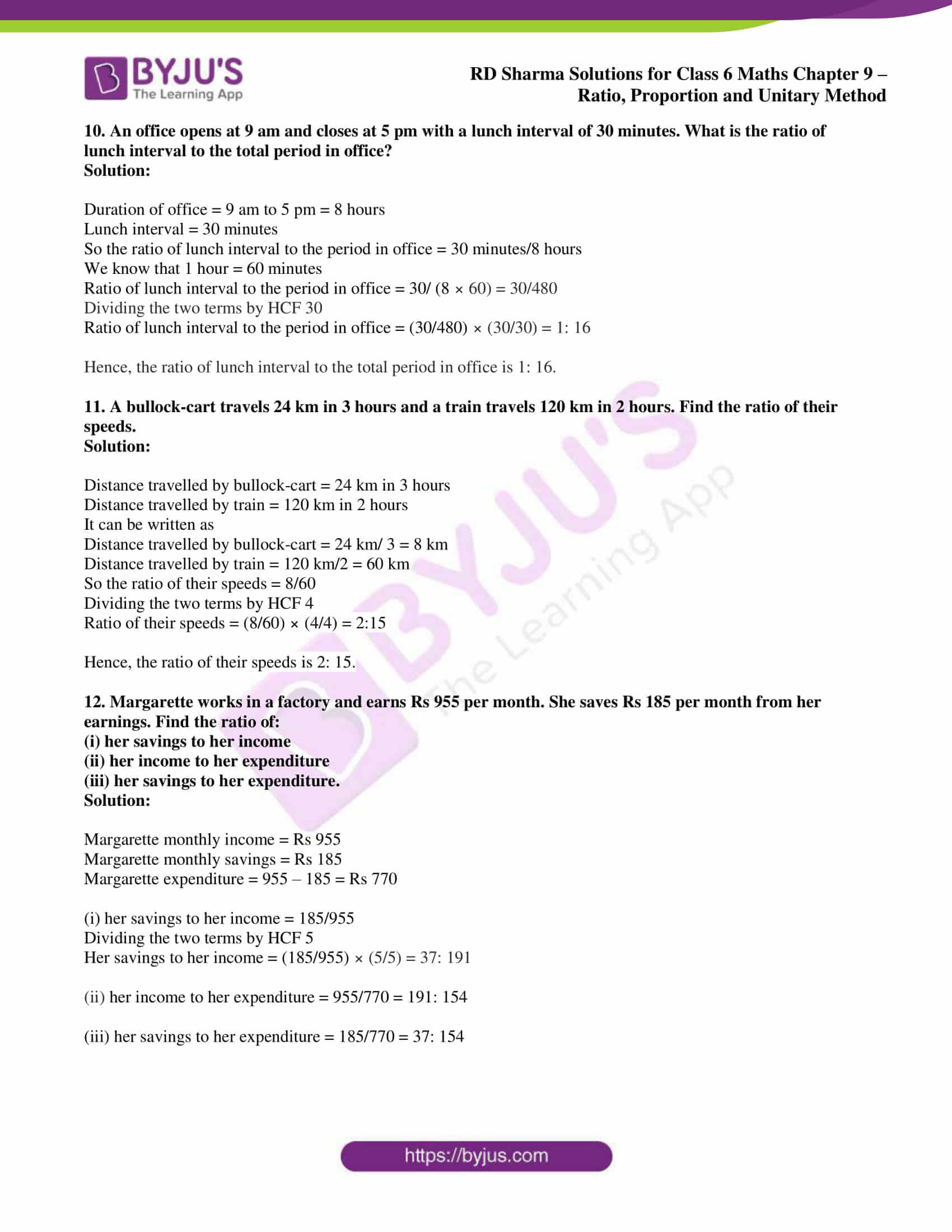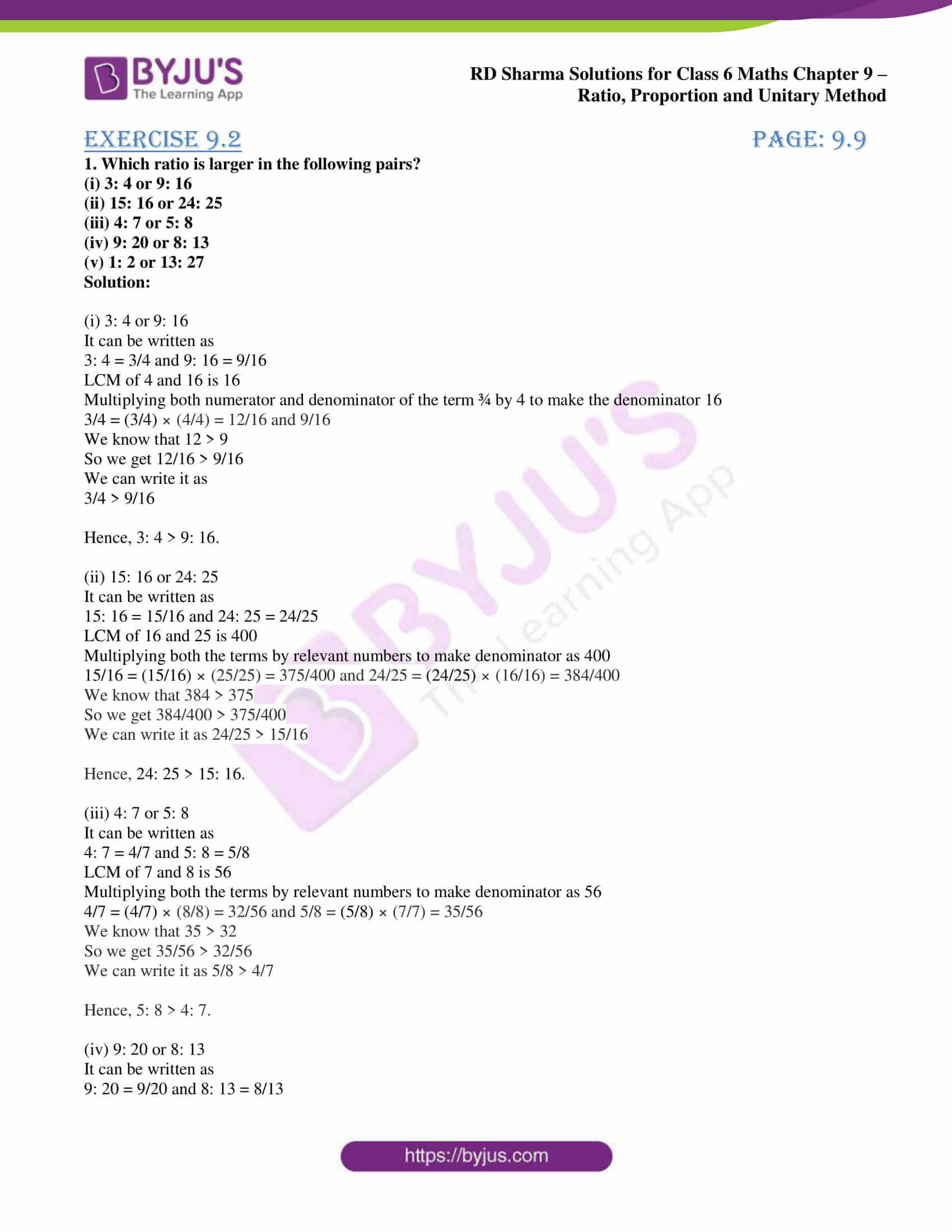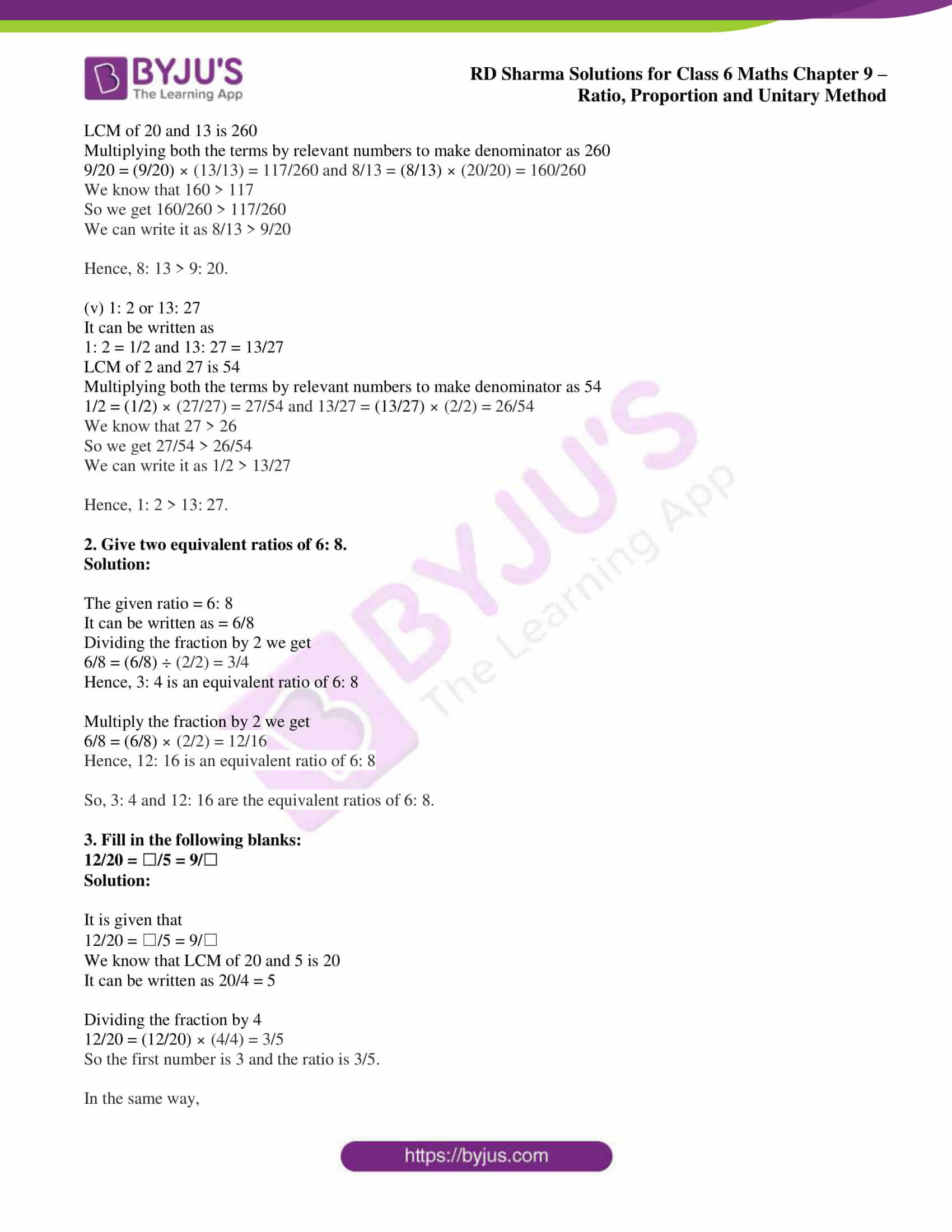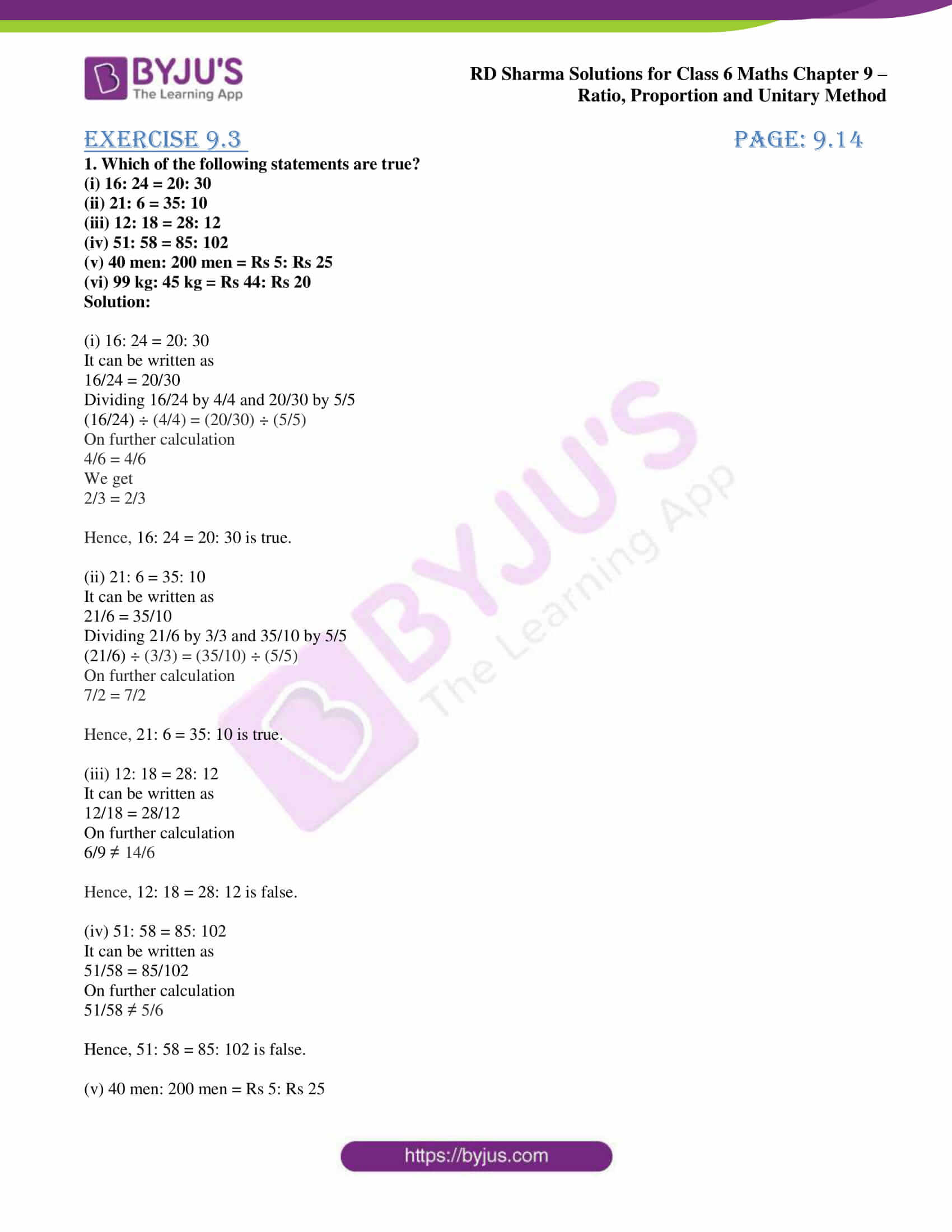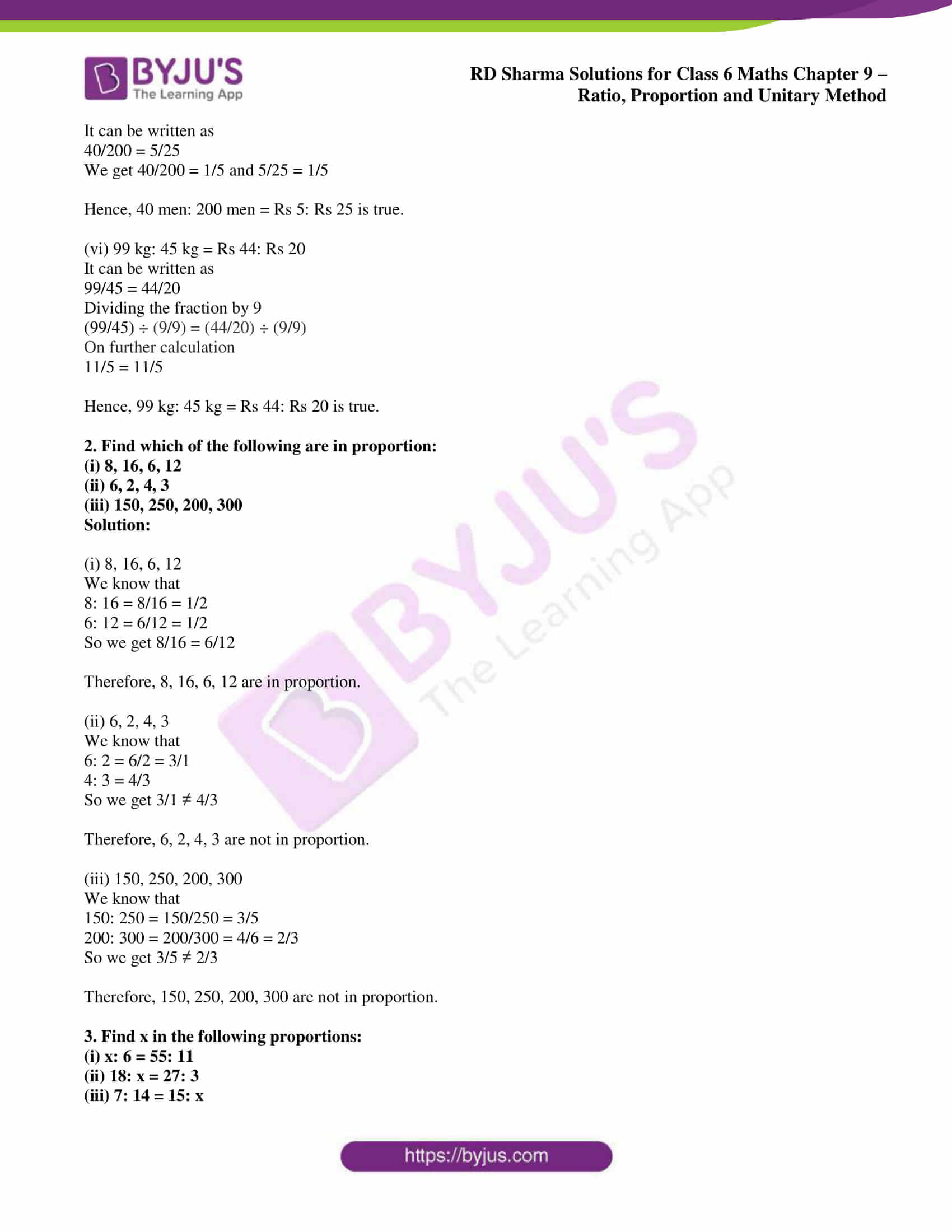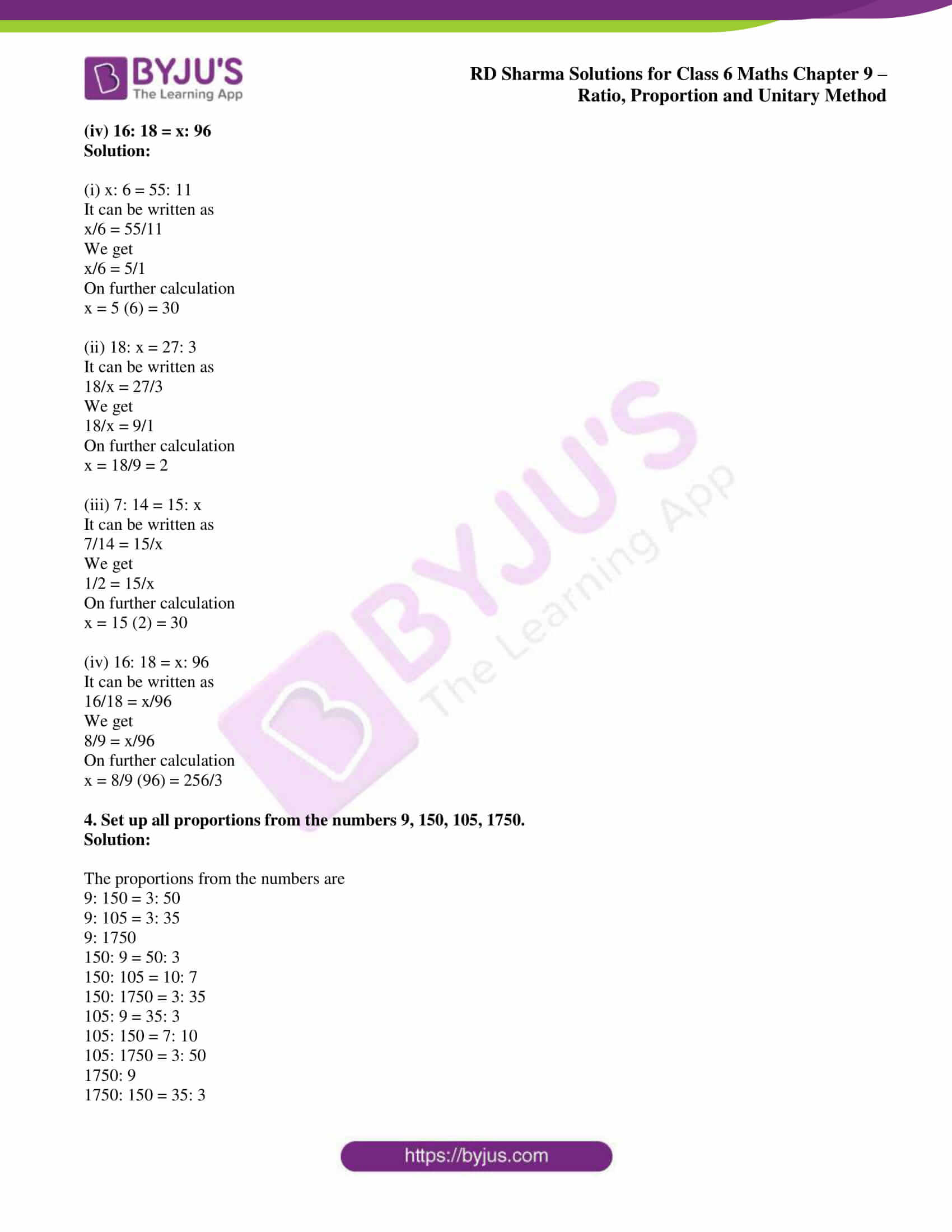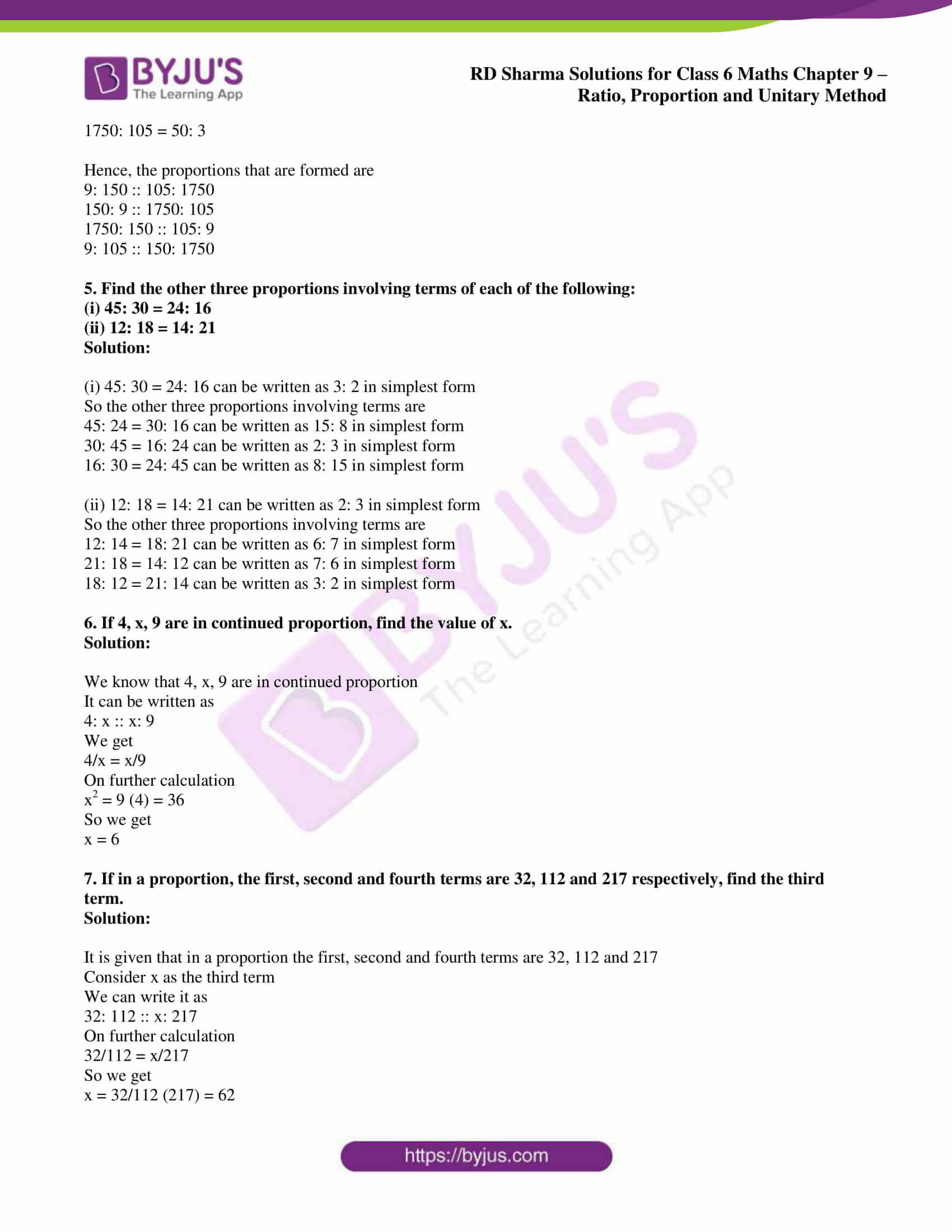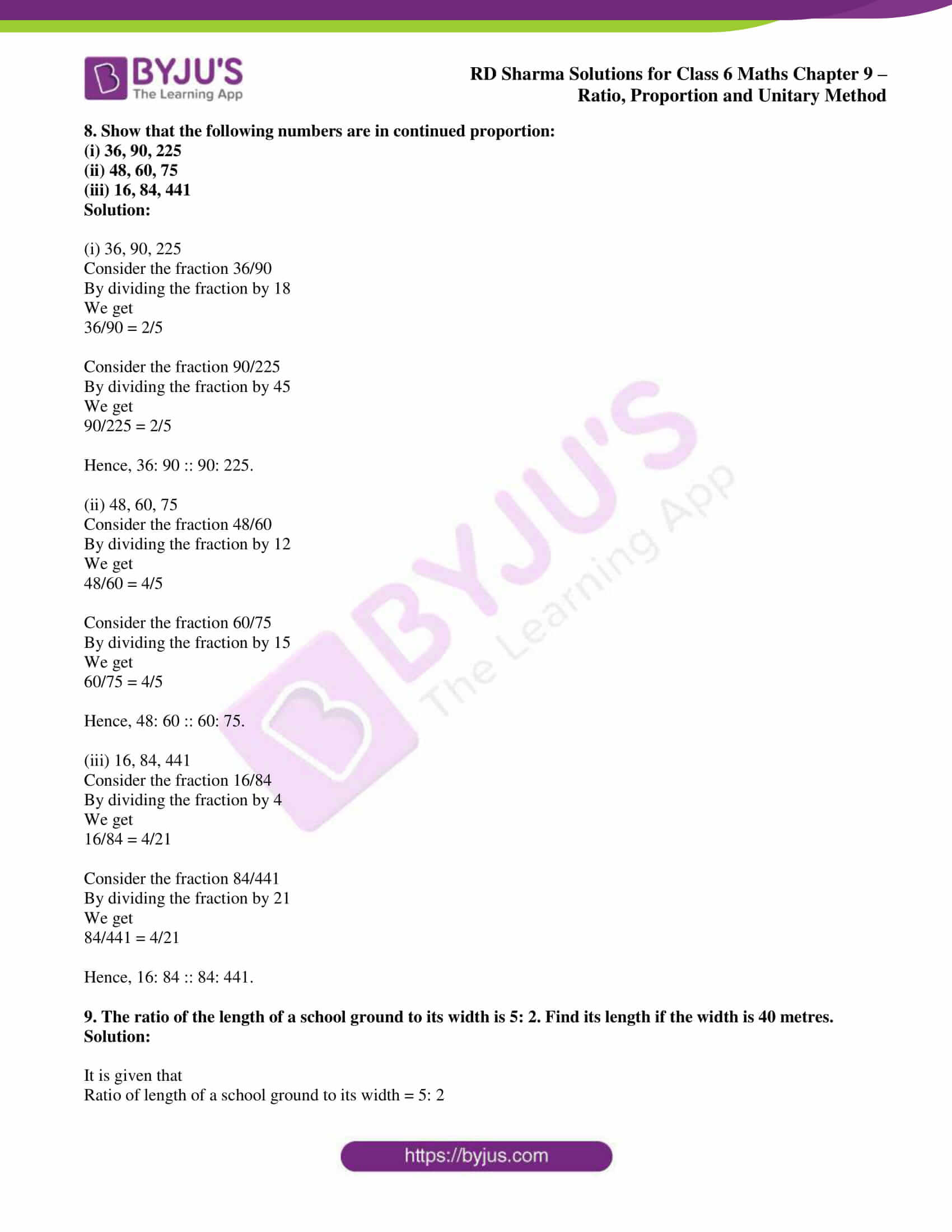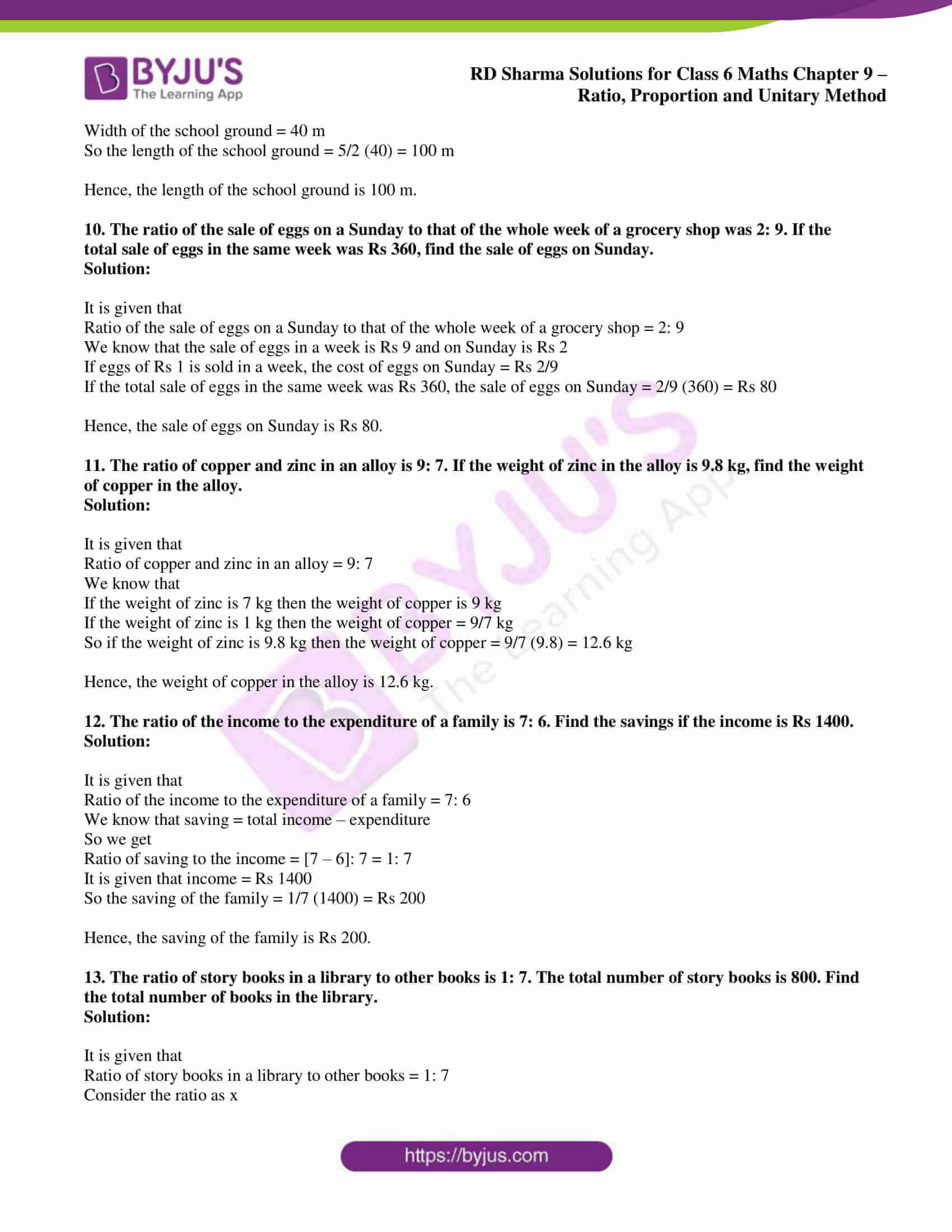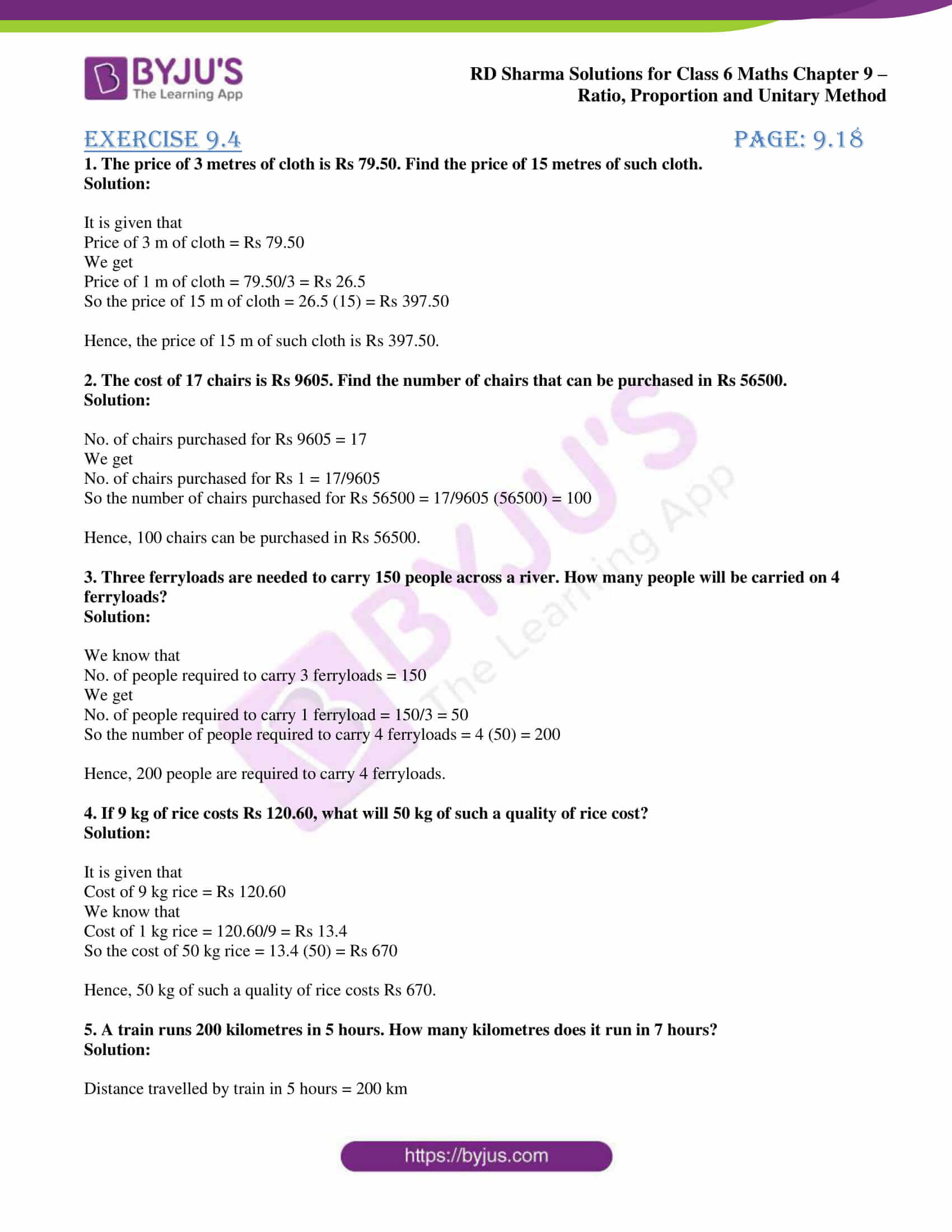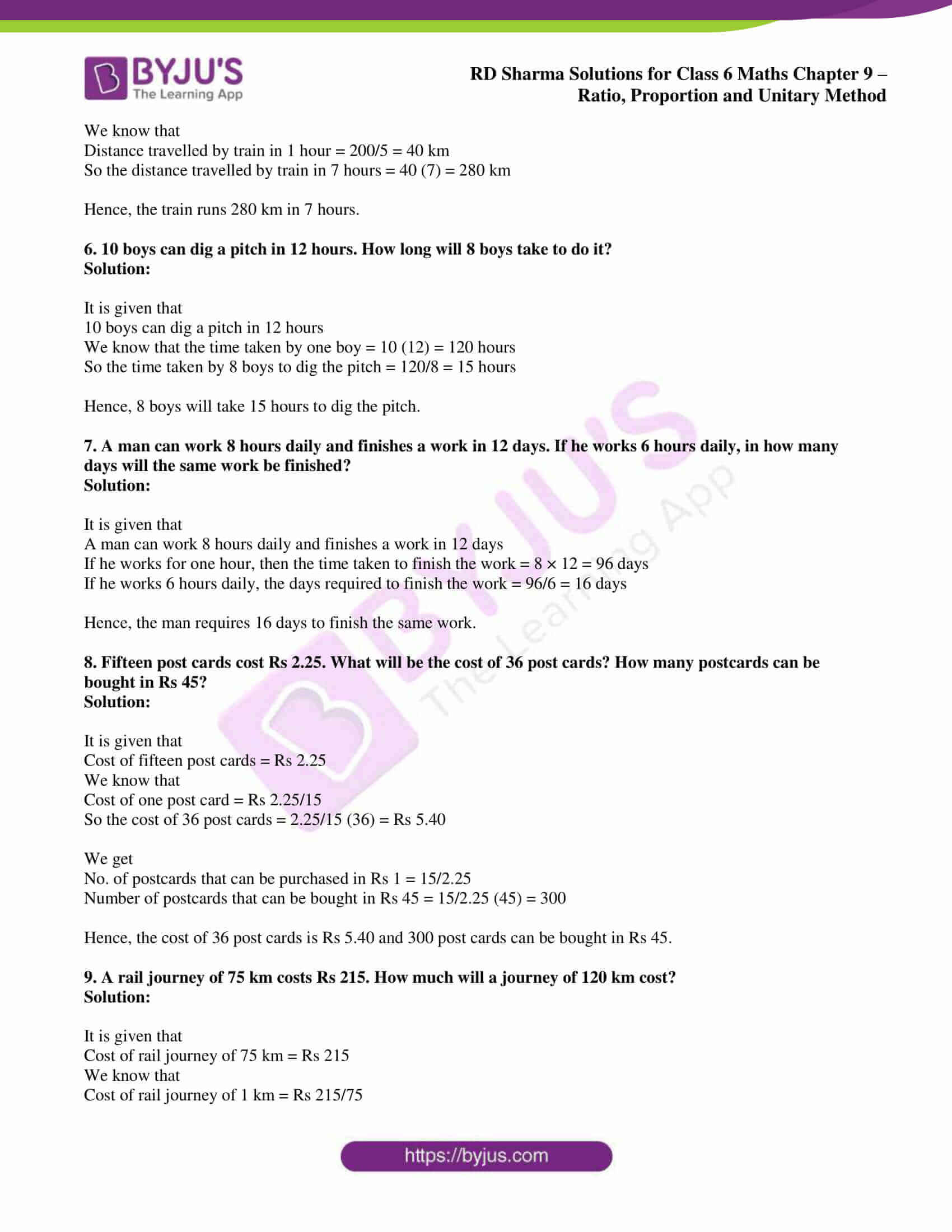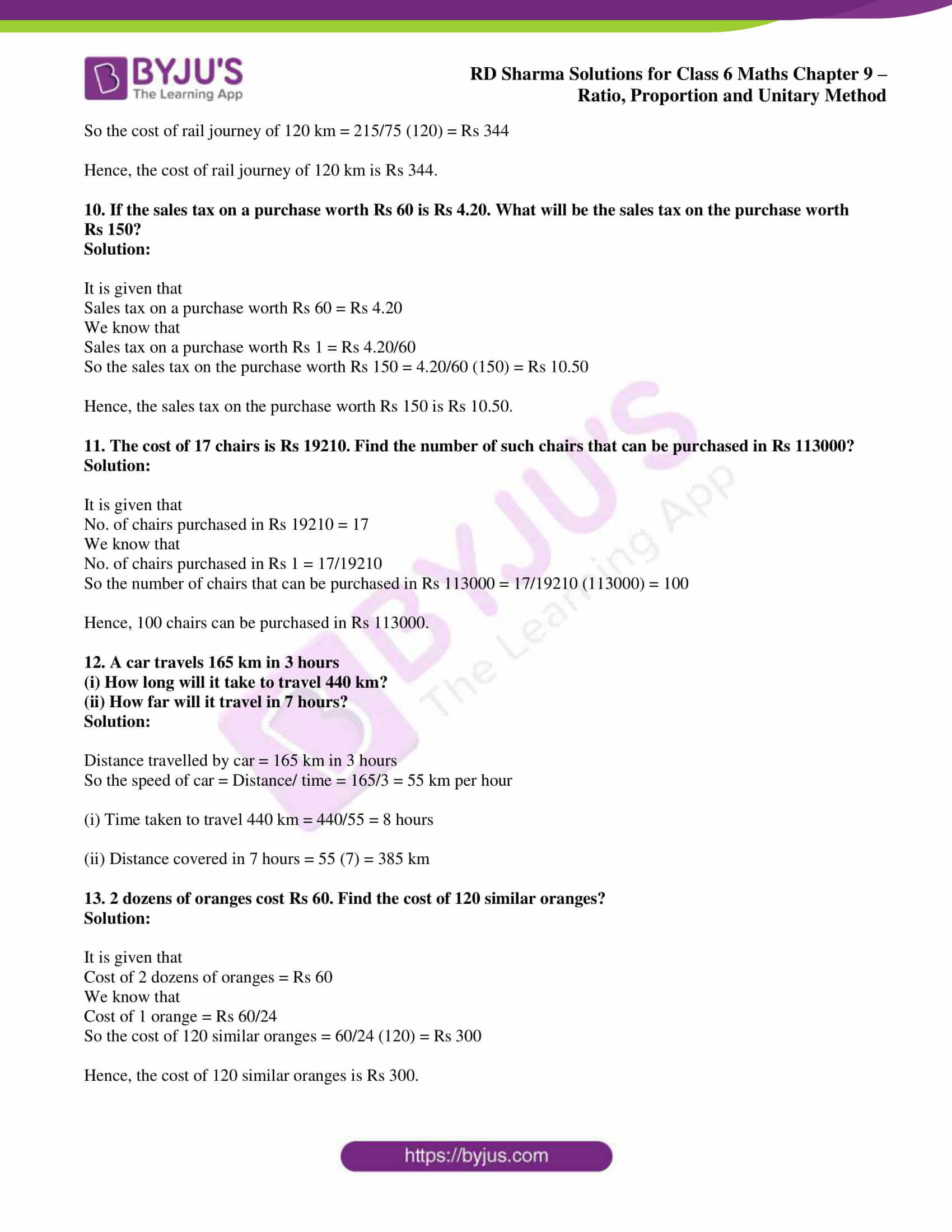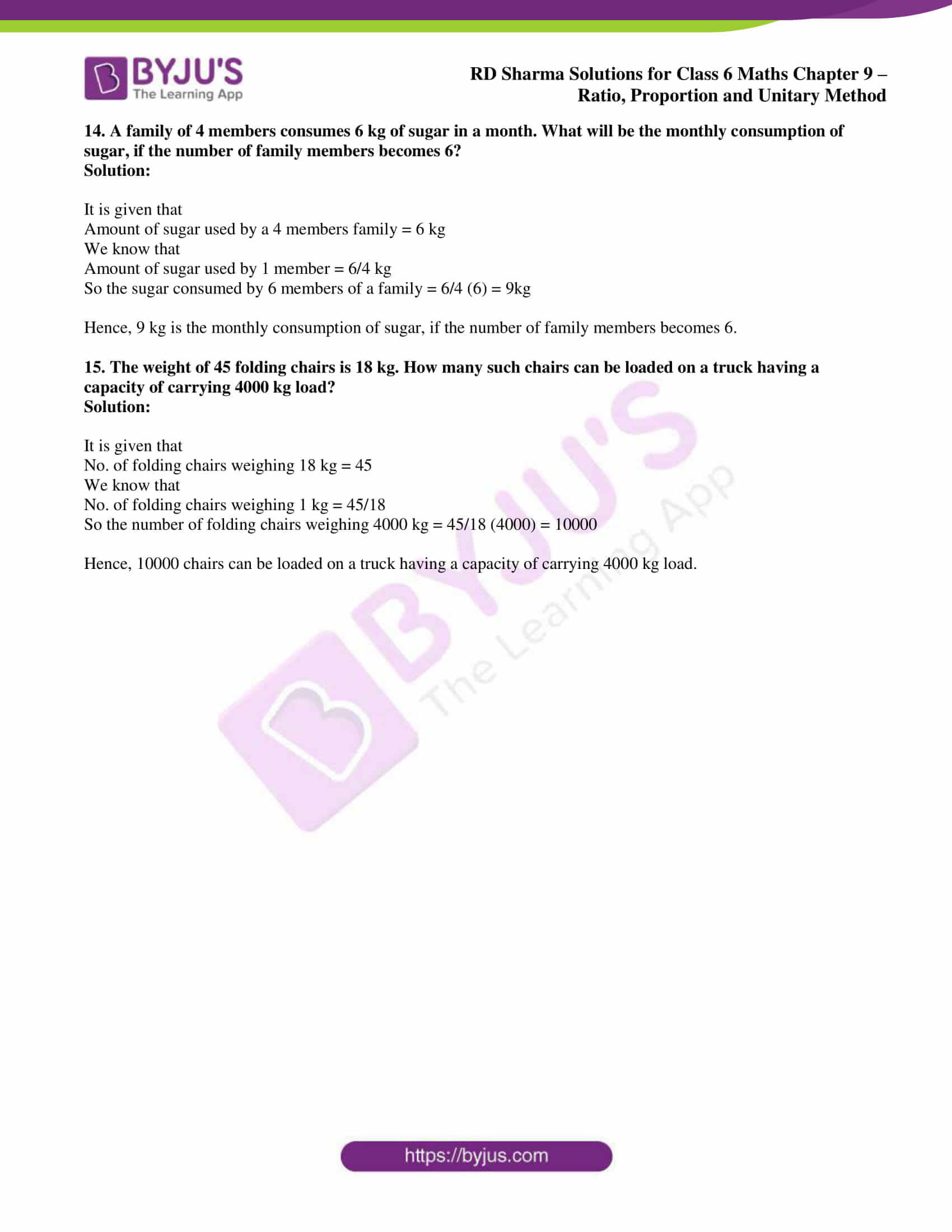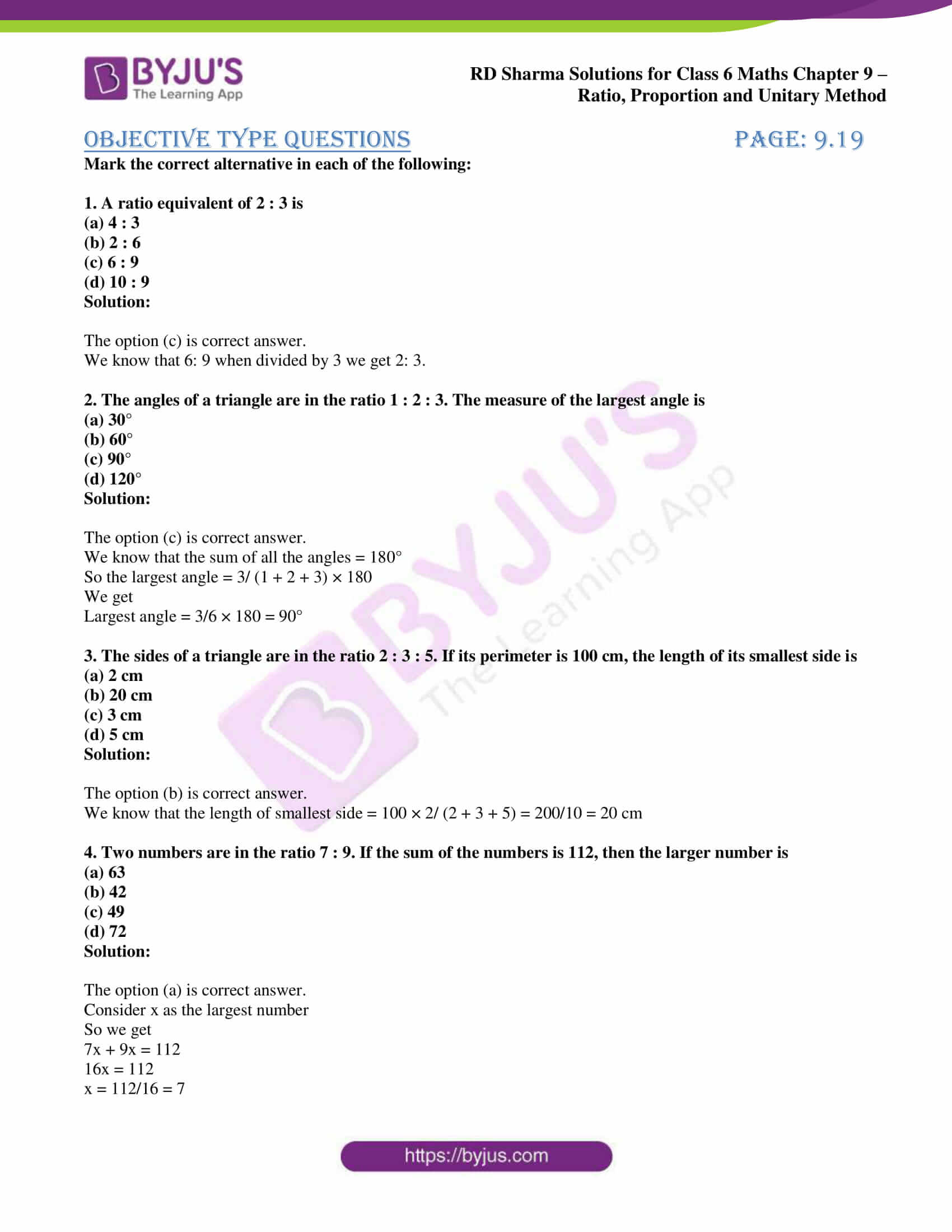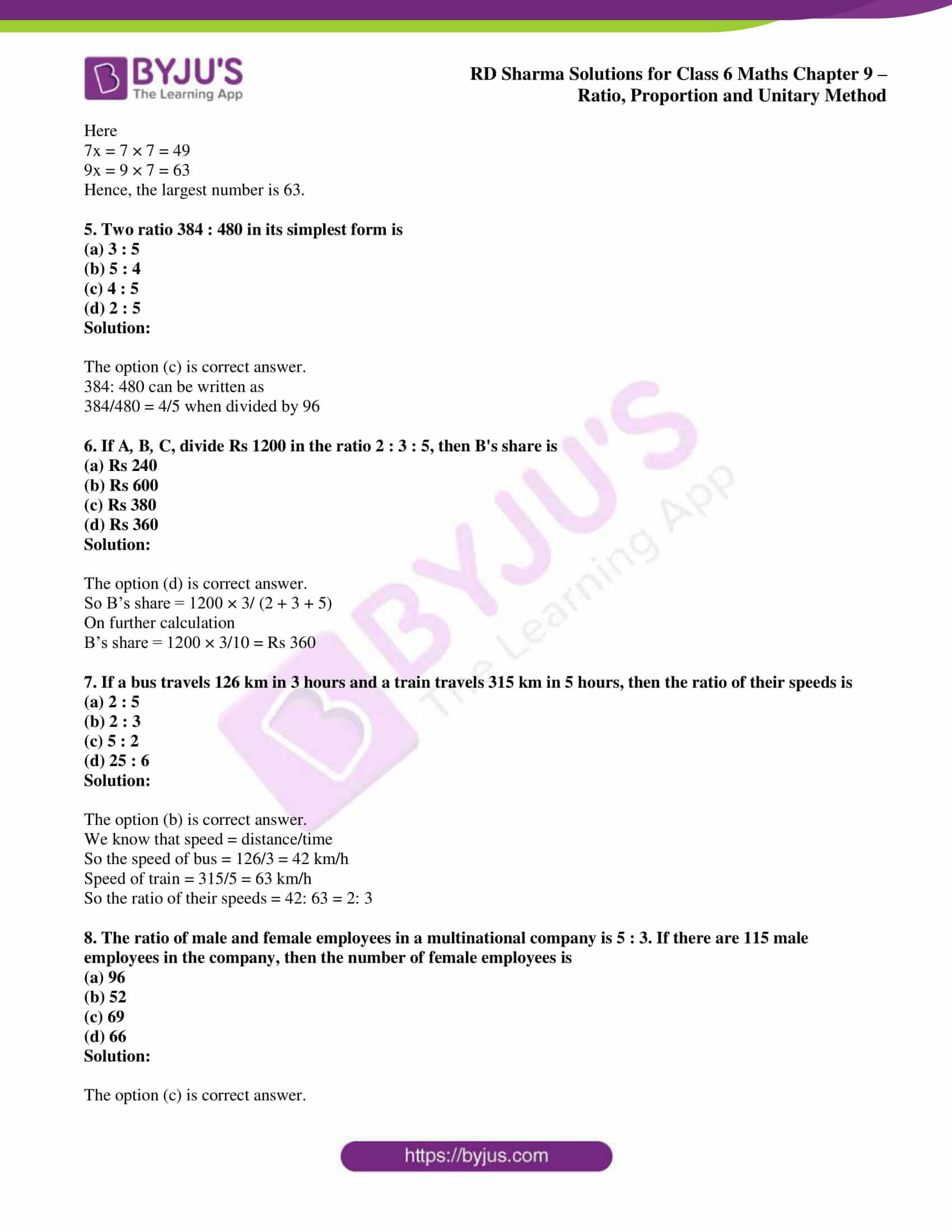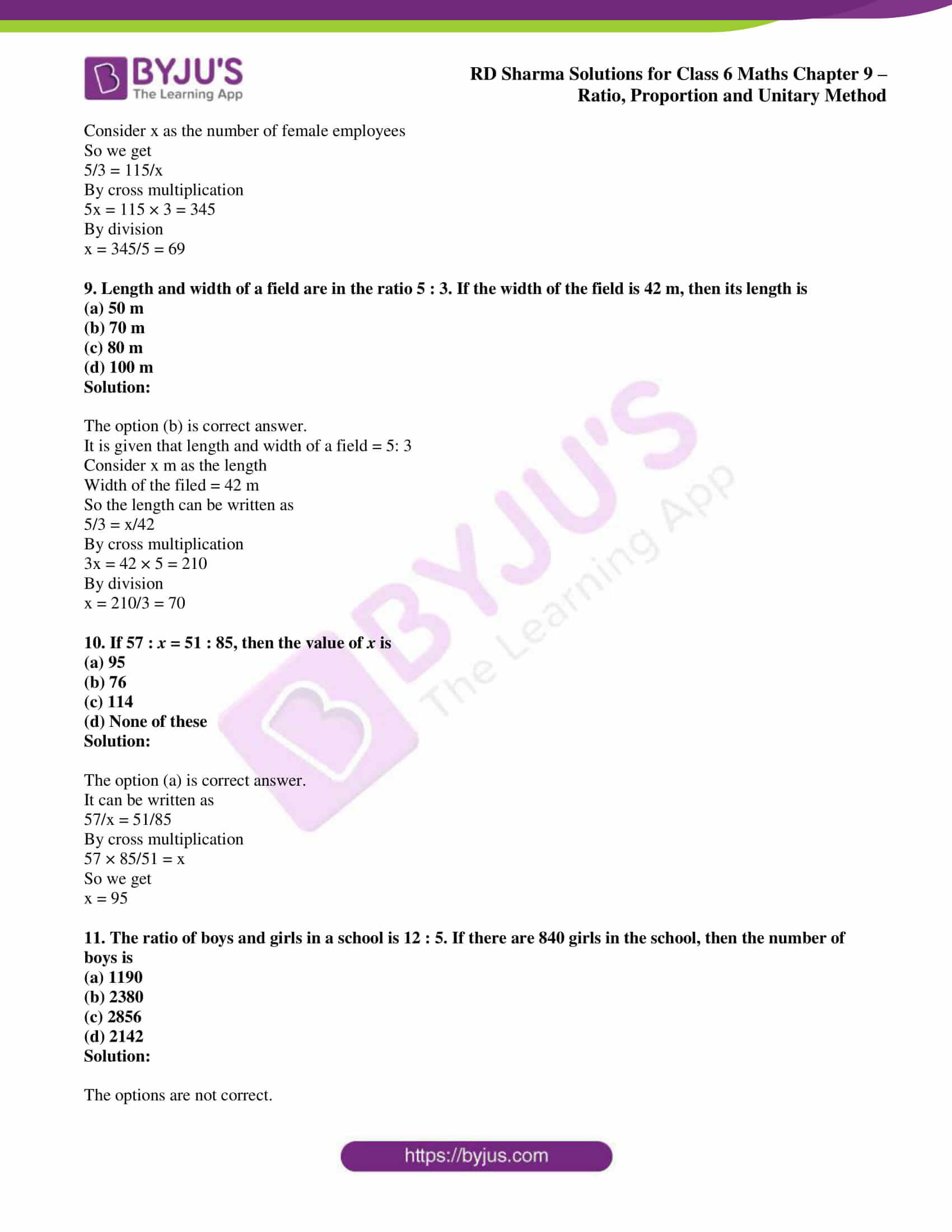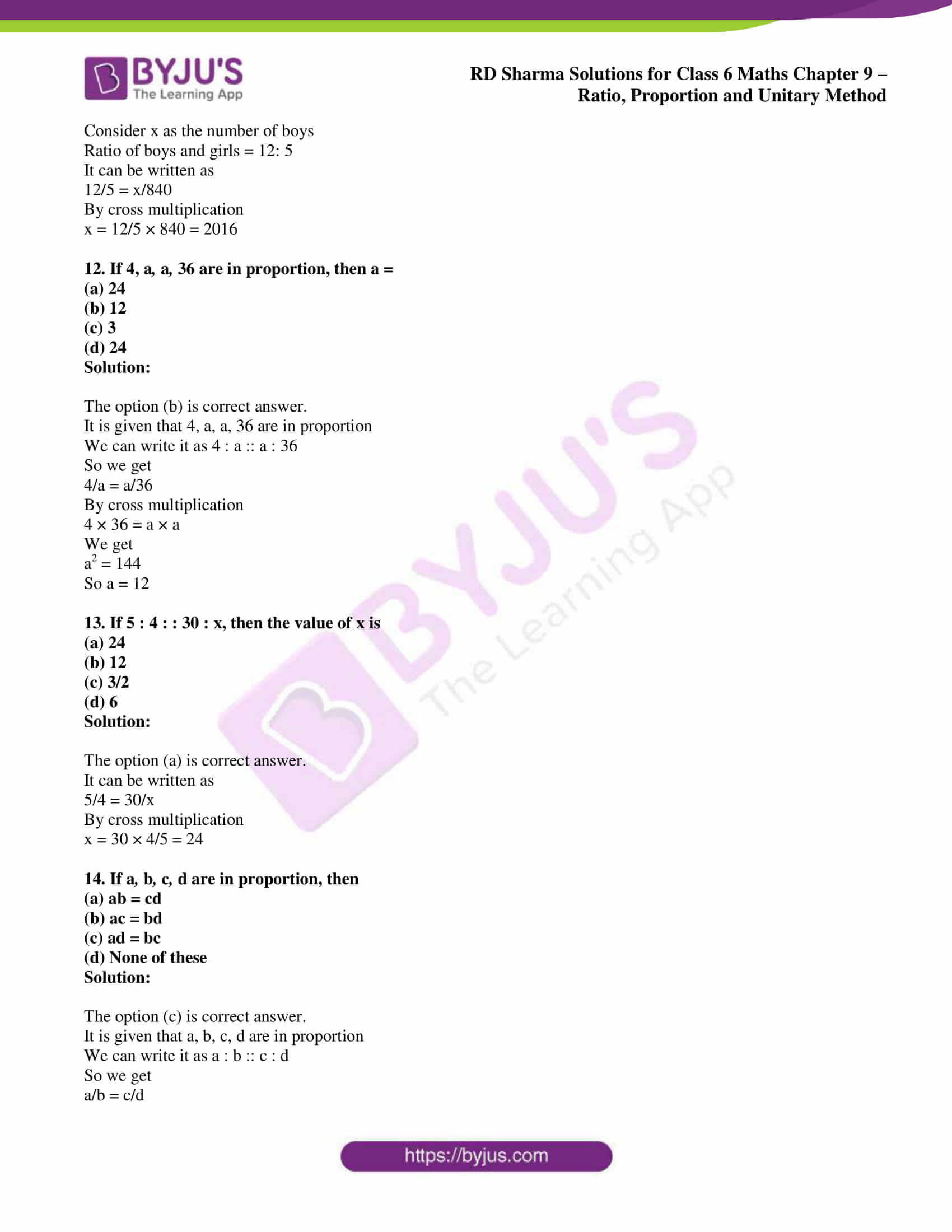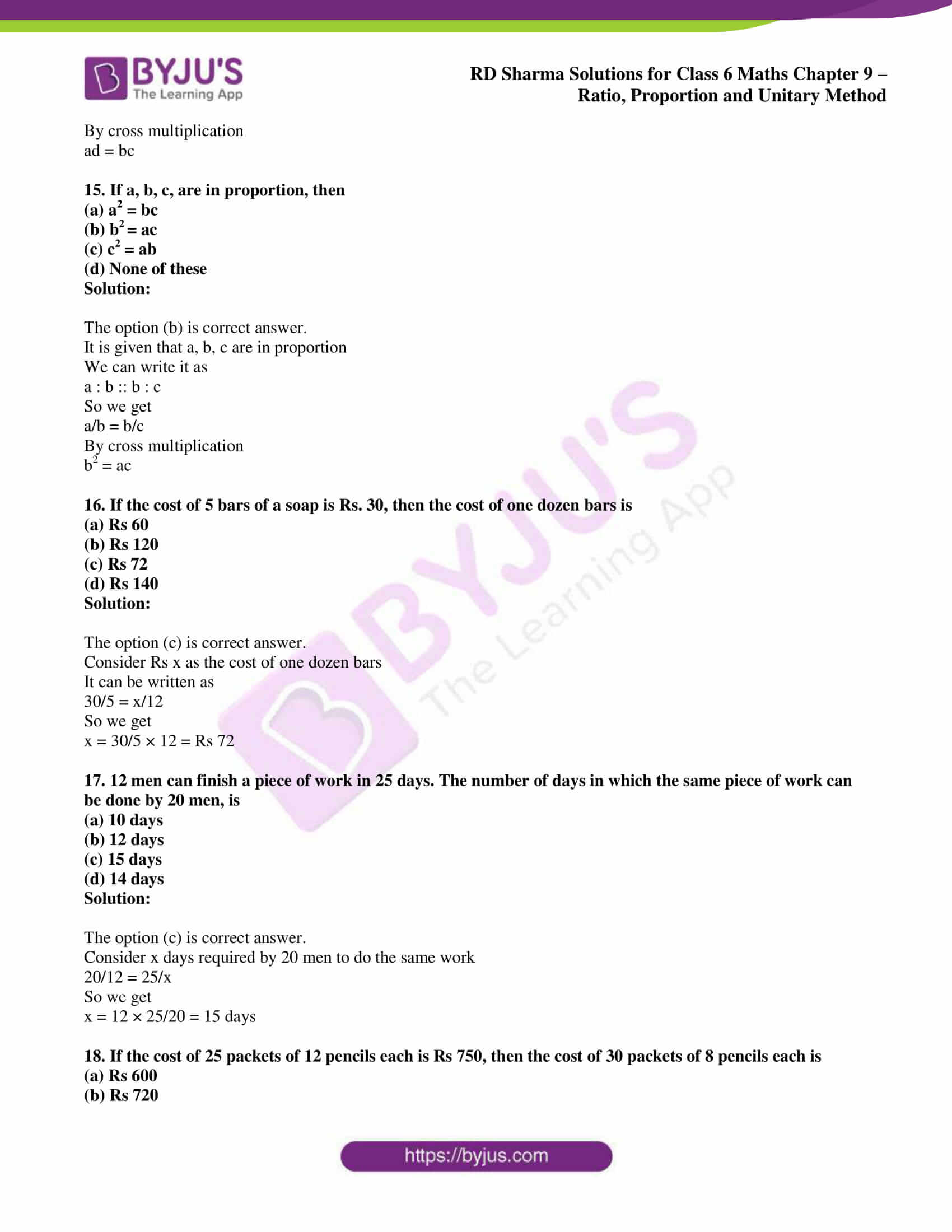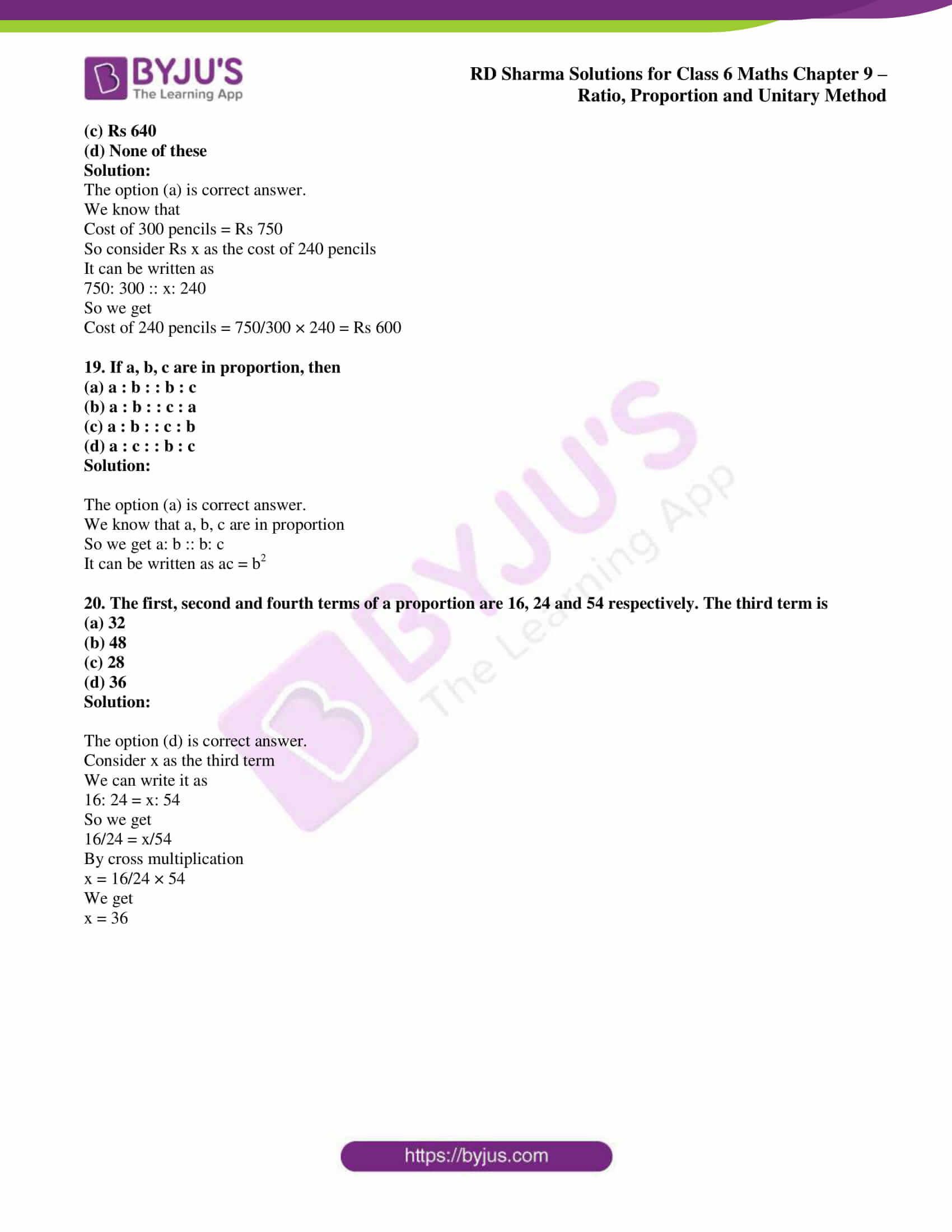## Access answers to Maths RD Sharma Solutions for Class 6 Chapter 9: Ratio, Proportion and Unitary Method

### Exercise 9.1 page: 9.5

1. Express each of the following in the language of ratios:

(i) In a class, the number of girls in the merit list of the board examination is two times that of boys.

(ii) The number of students passing mathematics test is 2/3 of the number that appeared.

Solution:

(i) Ratio of the number of girls to that of boys in the merit list is 2: 1.

(ii) Ratio of the number of students passing a mathematics test to that of total students appearing in the test is 2: 3.

2. Express the following ratios in language of daily life:

(i) The ratio of the number of bad pencils to that of good pencils produced in a factory is 1: 9.

(ii) In India, the ratio of the number of villages to that of cities is about 2000: 1.

Solution:

(i) The number of bad pencils produced in a factory is 1/9 of the number of good pencils produced in the factory.

(ii) The number of villages is 2000 times that of cities in India.

3. Express each of the following ratios in its simplest form:

(i) 60: 72

(ii) 324: 144

(iii) 85: 391

(iv) 186: 403

Solution:

(i) 60: 72

It can be written as 60/72

We know that the HCF of 60 and 72 is 12

By dividing the term by 12 we get

(60/72) × (12/12) = 5/6

So we get 60: 72 = 5: 6

(ii) 324: 144

It can be written as 324/144

We know that the HCF of 324 and 144 is 36

By dividing the term by 36 we get

(324/144) × (36/36) = 9/4

So we get 324: 144 = 9: 4

(iii) 85: 391

It can be written as 85/391

We know that the HCF of 85 and 391 is 17

By dividing the term by 17 we get

(85/391) × (17/17) = 5/23

So we get 85: 391 = 5: 23

(iv) 186: 403

It can be written as 186/403

We know that the HCF of 186 and 403 is 31

By dividing the term by 31 we get

(186/403) × (31/31) = 6/13

So we get 186: 403 = 6: 13

4. Find the ratio of the following in the simplest form:

(i) 75 paise to Rs 3

(ii) 35 minutes to 45 minutes

(iii) 8 kg to 400 gm

(iv) 48 minutes to 1 hour

(v) 2 metres to 35 cm

(vi) 35 minutes to 45 seconds

(vii) 2 dozen to 3 scores

(viii) 3 weeks to 3 days

(ix) 48 min to 2 hours 40 min

(x) 3 m 5 cm to 35 cm

Solution:

(i) 75 paise to Rs 3

It can be written as

75 paise to Rs 3 = 75 paise: Rs 3

We know that 1 Rs = 100 paise

So we get

75 paise to Rs 3 = 75 paise: 300 paise

Dividing the two terms by HCF 75

75 paise to Rs 3 = 1: 4

(ii) 35 minutes to 45 minutes

It can be written as

35 minutes to 45 minutes = 35 minutes: 45 minutes

Dividing the two terms by HCF 5

35 minutes to 45 minutes = 7: 9

(iii) 8 kg to 400 gm

It can be written as

8 kg to 400 gm = 8 kg: 400 gm

We know that 1 kg = 1000 gm

So we get

8 kg to 400 gm = 8000 gm: 400 gm

Dividing the two terms by HCF 400

8 kg to 400 gm = 20: 1

(iv) 48 minutes to 1 hour

It can be written as

48 minutes to 1 hour = 48 minutes: 1 hour

We know that 1 hour = 60 minutes

So we get

48 minutes to 1 hour = 48 minutes: 60 minutes

Dividing the two terms by HCF 12

48 minutes to 1 hour = 4: 5

(v) 2 metres to 35 cm

It can be written as

2 metres to 35 cm = 2 metres: 35 cm

We know that 1 m = 100 cm

So we get

2 metres to 35 cm = 200 cm: 35 cm

Dividing the two terms by HCF 5

2 metres to 35 cm = 40: 7

(vi) 35 minutes to 45 seconds

It can be written as

35 minutes to 45 seconds = 35 minutes: 45 seconds

We know that 1 minute = 60 seconds

So we get

35 minutes to 45 seconds = 2100 seconds: 45 seconds

Dividing the two terms by HCF 15

35 minutes to 45 seconds = 140: 3

(vii) 2 dozen to 3 scores

It can be written as

2 dozen to 3 scores = 2 dozen: 3 scores

We know that 1 dozen = 12 score = 20

So we get

2 dozen to 3 scores = 24: 60

Dividing the two terms by HCF 12

2 dozen to 3 scores = 2: 5

(viii) 3 weeks to 3 days

It can be written as

3 weeks to 3 days = 3 weeks: 3 days

We know that 1 week = 7 days

So we get

3 weeks to 3 days = 21 days: 3 days

Dividing the two terms by HCF 3

3 weeks to 3 days = 7: 1

(ix) 48 min to 2 hours 40 min

It can be written as

48 min to 2 hours 40 min = 48 min: 2 hours 40 min

We know that 1 hour = 60 minutes

So we get

48 min to 2 hours 40 min = 48 min: 160 min

Dividing the two terms by HCF 16

48 min to 2 hours 40 min = 3: 10

(x) 3 m 5 cm to 35 cm

It can be written as

3 m 5 cm to 35 cm = 3 m 5 cm: 35 cm

We know that 1 m = 100 cm

So we get

3 m 5 cm to 35 cm = 305 cm: 35 cm

Dividing the two terms by HCF 5

3 m 5 cm to 35 cm = 61: 7

5. Find the ratio of

(i) 3.2 metres to 56 metres

(ii) 10 metres to 25 cm

(iii) 25 paise to Rs 60

(iv) 10 litres to 0.25 litre

Solution:

(i) 3.2 metres to 56 metres

It can be written as

3.2 metres to 56 metres = 3.2 metres: 56 metres

Dividing the two terms by HCF 1.6

3.2 metres to 56 metres = 2: 35

(ii) 10 metres to 25 cm

It can be written as

10 metres to 25 cm = 10 m: 25 cm

We know that 1 m = 100 cm

10 metres to 25 cm = 1000 cm: 25 cm

Dividing the two terms by HCF 25

10 metres to 25 cm = 40: 1

(iii) 25 paise to Rs 60

It can be written as

25 paise to Rs 60 = 25 paise: Rs 60

We know that 1 Rs = 100 paise

25 paise to Rs 60 = 25 paise: 6000 paise

Dividing the two terms by HCF 25

25 paise to Rs 60 = 1: 240

(iv) 10 litres to 0.25 litre

It can be written as

10 litres to 0.25 litre = 10 litres: 0.25 litre

Dividing the two terms by HCF 0.25

10 litres to 0.25 litre = 40: 1

6. The number of boys and girls in a school are 1168 and 1095 respectively. Express the ratio of the number of boys to that of the girls in the simplest form.

Solution:

No. of boys = 1168

No. of girls = 1095

So the ratio of the number of boys to that of the girls = 1168: 1095

Dividing the two terms by HCF 73

Ratio of number of boys to that of the girls = 16: 15

Hence, the ratio of the number of boys to that of girls in simplest form is 16: 15.

7. Avinash works as a lecturer and earns Rs 12000 per month. His wife who is a doctor earns Rs 15000 per month. Find the following ratios:

(i) Avinash’s income to the income of his wife.

(ii) Avinash’s income to their total income.

Solution:

Avinash salary earned per month = Rs 12000

Avinash wife salary per month = Rs 15000

(i) Avinash’s income to the income of his wife = 12000/15000 = 4: 5

(ii) Avinash’s income to their total income = 12000/ (12000 + 15000) = 4: 9

8. Of the 72 persons working in an office, 28 are men and the remaining are women. Find the ratio of the number of:

(i) men to that of women,

(ii) men to the total number of persons

(iii) persons to that of women.

Solution:

No. of persons working in an office = 72

No. of men = 28

So the number of women = 72 – 28 = 44

(i) men to that of women = 28: 44

Multiplying and dividing the equation by HCF 4

Men to that of women = (28/44) × (4/4) = 7: 11

(ii) men to the total number of persons = 28: 72

Multiplying and dividing the equation by HCF 4

Men to the total number of persons = (28/72) × (4/4) = 7: 18

(iii) persons to that of women = 72: 44

Multiplying and dividing the equation by HCF 4

Persons to that of women = (72/44) × (4/4) = 18: 11

9. The length of a steel tape for measurements of buildings is 10 m and its width is 2.4 cm. What is the ratio of its length to width?

Solution:

It is given that

Length of a steel tape = 10 m

Width of steel tape = 2.4 cm

So the ratio of its length to width = 10 m/ 2.4 cm

We know that 1 m = 100 cm

Ratio of its length to width = 1000 cm/ 2.4 cm

Dividing the two terms by HCF 0.8 cm

Ratio of its length to width = 1250: 3

Hence, the ratio of its length to width is 1250: 3.

10. An office opens at 9 am and closes at 5 pm with a lunch interval of 30 minutes. What is the ratio of lunch interval to the total period in office?

Solution:

Duration of office = 9 am to 5 pm = 8 hours

Lunch interval = 30 minutes

So the ratio of lunch interval to the period in office = 30 minutes/8 hours

We know that 1 hour = 60 minutes

Ratio of lunch interval to the period in office = 30/ (8 × 60) = 30/480

Dividing the two terms by HCF 30

Ratio of lunch interval to the period in office = (30/480) × (30/30) = 1: 16

Hence, the ratio of lunch interval to the total period in office is 1: 16.

11. A bullock-cart travels 24 km in 3 hours and a train travels 120 km in 2 hours. Find the ratio of their speeds.

Solution:

Distance travelled by bullock-cart = 24 km in 3 hours

Distance travelled by train = 120 km in 2 hours

It can be written as

Distance travelled by bullock-cart = 24 km/ 3 = 8 km

Distance travelled by train = 120 km/2 = 60 km

So the ratio of their speeds = 8/60

Dividing the two terms by HCF 4

Ratio of their speeds = (8/60) × (4/4) = 2:15

Hence, the ratio of their speeds is 2: 15.

12. Margarette works in a factory and earns Rs 955 per month. She saves Rs 185 per month from her earnings. Find the ratio of:

(i) her savings to her income

(ii) her income to her expenditure

(iii) her savings to her expenditure.

Solution:

Margarette monthly income = Rs 955

Margarette monthly savings = Rs 185

Margarette expenditure = 955 – 185 = Rs 770

(i) her savings to her income = 185/955

Dividing the two terms by HCF 5

Her savings to her income = (185/955) × (5/5) = 37: 191

(ii) her income to her expenditure = 955/770 = 191: 154

(iii) her savings to her expenditure = 185/770 = 37: 154

### Exercise 9.2 page: 9.9

1. Which ratio is larger in the following pairs?

(i) 3: 4 or 9: 16

(ii) 15: 16 or 24: 25

(iii) 4: 7 or 5: 8

(iv) 9: 20 or 8: 13

(v) 1: 2 or 13: 27

Solution:

(i) 3: 4 or 9: 16

It can be written as

3: 4 = 3/4 and 9: 16 = 9/16

LCM of 4 and 16 is 16

Multiplying both numerator and denominator of the term ¾ by 4 to make the denominator 16

3/4 = (3/4) × (4/4) = 12/16 and 9/16

We know that 12 > 9

So we get 12/16 > 9/16

We can write it as

3/4 > 9/16

Hence, 3: 4 > 9: 16.

(ii) 15: 16 or 24: 25

It can be written as

15: 16 = 15/16 and 24: 25 = 24/25

LCM of 16 and 25 is 400

Multiplying both the terms by relevant numbers to make denominator as 400

15/16 = (15/16) × (25/25) = 375/400 and 24/25 = (24/25) × (16/16) = 384/400

We know that 384 > 375

So we get 384/400 > 375/400

We can write it as 24/25 > 15/16

Hence, 24: 25 > 15: 16.

(iii) 4: 7 or 5: 8

It can be written as

4: 7 = 4/7 and 5: 8 = 5/8

LCM of 7 and 8 is 56

Multiplying both the terms by relevant numbers to make denominator as 56

4/7 = (4/7) × (8/8) = 32/56 and 5/8 = (5/8) × (7/7) = 35/56

We know that 35 > 32

So we get 35/56 > 32/56

We can write it as 5/8 > 4/7

Hence, 5: 8 > 4: 7.

(iv) 9: 20 or 8: 13

It can be written as

9: 20 = 9/20 and 8: 13 = 8/13

LCM of 20 and 13 is 260

Multiplying both the terms by relevant numbers to make denominator as 260

9/20 = (9/20) × (13/13) = 117/260 and 8/13 = (8/13) × (20/20) = 160/260

We know that 160 > 117

So we get 160/260 > 117/260

We can write it as 8/13 > 9/20

Hence, 8: 13 > 9: 20.

(v) 1: 2 or 13: 27

It can be written as

1: 2 = 1/2 and 13: 27 = 13/27

LCM of 2 and 27 is 54

Multiplying both the terms by relevant numbers to make denominator as 54

1/2 = (1/2) × (27/27) = 27/54 and 13/27 = (13/27) × (2/2) = 26/54

We know that 27 > 26

So we get 27/54 > 26/54

We can write it as 1/2 > 13/27

Hence, 1: 2 > 13: 27.

2. Give two equivalent ratios of 6: 8.

Solution:

The given ratio = 6: 8

It can be written as = 6/8

Dividing the fraction by 2 we get

6/8 = (6/8) ÷ (2/2) = 3/4

Hence, 3: 4 is an equivalent ratio of 6: 8

Multiply the fraction by 2 we get

6/8 = (6/8) × (2/2) = 12/16

Hence, 12: 16 is an equivalent ratio of 6: 8

So, 3: 4 and 12: 16 are the equivalent ratios of 6: 8.

3. Fill in the following blanks:

12/20 = ☐/5 = 9/☐

Solution:

It is given that

12/20 = ☐/5 = 9/☐

We know that LCM of 20 and 5 is 20

It can be written as 20/4 = 5

Dividing the fraction by 4

12/20 = (12/20) × (4/4) = 3/5

So the first number is 3 and the ratio is 3/5.

In the same way,

Consider 2/3 + 3/5 = 9/☐

We know that 9/3 = 3

Multiply the fraction by 3

3/5 = (3/5) × (3/3) = 9/15

So the second number is 15 and the ratio is 9/15.

### Exercise 9.3 page: 9.14

1. Which of the following statements are true?

(i) 16: 24 = 20: 30

(ii) 21: 6 = 35: 10

(iii) 12: 18 = 28: 12

(iv) 51: 58 = 85: 102

(v) 40 men: 200 men = Rs 5: Rs 25

(vi) 99 kg: 45 kg = Rs 44: Rs 20

Solution:

(i) 16: 24 = 20: 30

It can be written as

16/24 = 20/30

Dividing 16/24 by 4/4 and 20/30 by 5/5

(16/24) ÷ (4/4) = (20/30) ÷ (5/5)

On further calculation

4/6 = 4/6

We get

2/3 = 2/3

Hence, 16: 24 = 20: 30 is true.

(ii) 21: 6 = 35: 10

It can be written as

21/6 = 35/10

Dividing 21/6 by 3/3 and 35/10 by 5/5

(21/6) ÷ (3/3) = (35/10) ÷ (5/5)

On further calculation

7/2 = 7/2

Hence, 21: 6 = 35: 10 is true.

(iii) 12: 18 = 28: 12

It can be written as

12/18 = 28/12

On further calculation

6/9 ≠ 14/6

Hence, 12: 18 = 28: 12 is false.

(iv) 51: 58 = 85: 102

It can be written as

51/58 = 85/102

On further calculation

51/58 ≠ 5/6

Hence, 51: 58 = 85: 102 is false.

(v) 40 men: 200 men = Rs 5: Rs 25

It can be written as

40/200 = 5/25

We get 40/200 = 1/5 and 5/25 = 1/5

Hence, 40 men: 200 men = Rs 5: Rs 25 is true.

(vi) 99 kg: 45 kg = Rs 44: Rs 20

It can be written as

99/45 = 44/20

Dividing the fraction by 9

(99/45) ÷ (9/9) = (44/20) ÷ (9/9)

On further calculation

11/5 = 11/5

Hence, 99 kg: 45 kg = Rs 44: Rs 20 is true.

2. Find which of the following are in proportion:

(i) 8, 16, 6, 12

(ii) 6, 2, 4, 3

(iii) 150, 250, 200, 300

Solution:

(i) 8, 16, 6, 12

We know that

8: 16 = 8/16 = 1/2

6: 12 = 6/12 = 1/2

So we get 8/16 = 6/12

Therefore, 8, 16, 6, 12 are in proportion.

(ii) 6, 2, 4, 3

We know that

6: 2 = 6/2 = 3/1

4: 3 = 4/3

So we get 3/1 ≠ 4/3

Therefore, 6, 2, 4, 3 are not in proportion.

(iii) 150, 250, 200, 300

We know that

150: 250 = 150/250 = 3/5

200: 300 = 200/300 = 4/6 = 2/3

So we get 3/5 ≠ 2/3

Therefore, 150, 250, 200, 300 are not in proportion.

3. Find x in the following proportions:

(i) x: 6 = 55: 11

(ii) 18: x = 27: 3

(iii) 7: 14 = 15: x

(iv) 16: 18 = x: 96

Solution:

(i) x: 6 = 55: 11

It can be written as

x/6 = 55/11

We get

x/6 = 5/1

On further calculation

x = 5 (6) = 30

(ii) 18: x = 27: 3

It can be written as

18/x = 27/3

We get

18/x = 9/1

On further calculation

x = 18/9 = 2

(iii) 7: 14 = 15: x

It can be written as

7/14 = 15/x

We get

1/2 = 15/x

On further calculation

x = 15 (2) = 30

(iv) 16: 18 = x: 96

It can be written as

16/18 = x/96

We get

8/9 = x/96

On further calculation

x = 8/9 (96) = 256/3

4. Set up all proportions from the numbers 9, 150, 105, 1750.

Solution:

The proportions from the numbers are

9: 150 = 3: 50

9: 105 = 3: 35

9: 1750

150: 9 = 50: 3

150: 105 = 10: 7

150: 1750 = 3: 35

105: 9 = 35: 3

105: 150 = 7: 10

105: 1750 = 3: 50

1750: 9

1750: 150 = 35: 3

1750: 105 = 50: 3

Hence, the proportions that are formed are

9: 150 :: 105: 1750

150: 9 :: 1750: 105

1750: 150 :: 105: 9

9: 105 :: 150: 1750

5. Find the other three proportions involving terms of each of the following:

(i) 45: 30 = 24: 16

(ii) 12: 18 = 14: 21

Solution:

(i) 45: 30 = 24: 16 can be written as 3: 2 in simplest form

So the other three proportions involving terms are

45: 24 = 30: 16 can be written as 15: 8 in simplest form

30: 45 = 16: 24 can be written as 2: 3 in simplest form

16: 30 = 24: 45 can be written as 8: 15 in simplest form

(ii) 12: 18 = 14: 21 can be written as 2: 3 in simplest form

So the other three proportions involving terms are

12: 14 = 18: 21 can be written as 6: 7 in simplest form

21: 18 = 14: 12 can be written as 7: 6 in simplest form

18: 12 = 21: 14 can be written as 3: 2 in simplest form

6. If 4, x, 9 are in continued proportion, find the value of x.

Solution:

We know that 4, x, 9 are in continued proportion

It can be written as

4: x :: x: 9

We get

4/x = x/9

On further calculation

x2 = 9 (4) = 36

So we get

x = 6

7. If in a proportion, the first, second and fourth terms are 32, 112 and 217 respectively, find the third term.

Solution:

It is given that in a proportion the first, second and fourth terms are 32, 112 and 217

Consider x as the third term

We can write it as

32: 112 :: x: 217

On further calculation

32/112 = x/217

So we get

x = 32/112 (217) = 62

8. Show that the following numbers are in continued proportion:

(i) 36, 90, 225

(ii) 48, 60, 75

(iii) 16, 84, 441

Solution:

(i) 36, 90, 225

Consider the fraction 36/90

By dividing the fraction by 18

We get

36/90 = 2/5

Consider the fraction 90/225

By dividing the fraction by 45

We get

90/225 = 2/5

Hence, 36: 90 :: 90: 225.

(ii) 48, 60, 75

Consider the fraction 48/60

By dividing the fraction by 12

We get

48/60 = 4/5

Consider the fraction 60/75

By dividing the fraction by 15

We get

60/75 = 4/5

Hence, 48: 60 :: 60: 75.

(iii) 16, 84, 441

Consider the fraction 16/84

By dividing the fraction by 4

We get

16/84 = 4/21

Consider the fraction 84/441

By dividing the fraction by 21

We get

84/441 = 4/21

Hence, 16: 84 :: 84: 441.

9. The ratio of the length of a school ground to its width is 5: 2. Find its length if the width is 40 metres.

Solution:

It is given that

Ratio of length of a school ground to its width = 5: 2

Width of the school ground = 40 m

So the length of the school ground = 5/2 (40) = 100 m

Hence, the length of the school ground is 100 m.

10. The ratio of the sale of eggs on a Sunday to that of the whole week of a grocery shop was 2: 9. If the total sale of eggs in the same week was Rs 360, find the sale of eggs on Sunday.

Solution:

It is given that

Ratio of the sale of eggs on a Sunday to that of the whole week of a grocery shop = 2: 9

We know that the sale of eggs in a week is Rs 9 and on Sunday is Rs 2

If eggs of Rs 1 is sold in a week, the cost of eggs on Sunday = Rs 2/9

If the total sale of eggs in the same week was Rs 360, the sale of eggs on Sunday = 2/9 (360) = Rs 80

Hence, the sale of eggs on Sunday is Rs 80.

11. The ratio of copper and zinc in an alloy is 9: 7. If the weight of zinc in the alloy is 9.8 kg, find the weight of copper in the alloy.

Solution:

It is given that

Ratio of copper and zinc in an alloy = 9: 7

We know that

If the weight of zinc is 7 kg then the weight of copper is 9 kg

If the weight of zinc is 1 kg then the weight of copper = 9/7 kg

So if the weight of zinc is 9.8 kg then the weight of copper = 9/7 (9.8) = 12.6 kg

Hence, the weight of copper in the alloy is 12.6 kg.

12. The ratio of the income to the expenditure of a family is 7: 6. Find the savings if the income is Rs 1400.

Solution:

It is given that

Ratio of the income to the expenditure of a family = 7: 6

We know that saving = total income – expenditure

So we get

Ratio of saving to the income = [7 – 6]: 7 = 1: 7

It is given that income = Rs 1400

So the saving of the family = 1/7 (1400) = Rs 200

Hence, the saving of the family is Rs 200.

13. The ratio of story books in a library to other books is 1: 7. The total number of story books is 800. Find the total number of books in the library.

Solution:

It is given that

Ratio of story books in a library to other books = 1: 7

Consider the ratio as x

So the number of story books = x

Number of other books = 7x

We know that

Total number of story books = 800

Number of other books = 7 × 800 = 5600

Total number of books = 5600 + 800 = 6400

Hence, the total number of books in the library is 6400.

### Exercise 9.4 PAGE: 9.18

1. The price of 3 metres of cloth is Rs 79.50. Find the price of 15 metres of such cloth.

Solution:

It is given that

Price of 3 m of cloth = Rs 79.50

We get

Price of 1 m of cloth = 79.50/3 = Rs 26.5

So the price of 15 m of cloth = 26.5 (15) = Rs 397.50

Hence, the price of 15 m of such cloth is Rs 397.50.

2. The cost of 17 chairs is Rs 9605. Find the number of chairs that can be purchased in Rs 56500.

Solution:

No. of chairs purchased for Rs 9605 = 17

We get

No. of chairs purchased for Rs 1 = 17/9605

So the number of chairs purchased for Rs 56500 = 17/9605 (56500) = 100

Hence, 100 chairs can be purchased in Rs 56500.

3. Three ferryloads are needed to carry 150 people across a river. How many people will be carried on 4 ferryloads?

Solution:

We know that

No. of people required to carry 3 ferryloads = 150

We get

No. of people required to carry 1 ferryload = 150/3 = 50

So the number of people required to carry 4 ferryloads = 4 (50) = 200

Hence, 200 people are required to carry 4 ferryloads.

4. If 9 kg of rice costs Rs 120.60, what will 50 kg of such a quality of rice cost?

Solution:

It is given that

Cost of 9 kg rice = Rs 120.60

We know that

Cost of 1 kg rice = 120.60/9 = Rs 13.4

So the cost of 50 kg rice = 13.4 (50) = Rs 670

Hence, 50 kg of such a quality of rice costs Rs 670.

5. A train runs 200 kilometres in 5 hours. How many kilometres does it run in 7 hours?

Solution:

Distance travelled by train in 5 hours = 200 km

We know that

Distance travelled by train in 1 hour = 200/5 = 40 km

So the distance travelled by train in 7 hours = 40 (7) = 280 km

Hence, the train runs 280 km in 7 hours.

6. 10 boys can dig a pitch in 12 hours. How long will 8 boys take to do it?

Solution:

It is given that

10 boys can dig a pitch in 12 hours

We know that the time taken by one boy = 10 (12) = 120 hours

So the time taken by 8 boys to dig the pitch = 120/8 = 15 hours

Hence, 8 boys will take 15 hours to dig the pitch.

7. A man can work 8 hours daily and finishes a work in 12 days. If he works 6 hours daily, in how many days will the same work be finished?

Solution:

It is given that

A man can work 8 hours daily and finishes a work in 12 days

If he works for one hour, then the time taken to finish the work = 8 × 12 = 96 days

If he works 6 hours daily, the days required to finish the work = 96/6 = 16 days

Hence, the man requires 16 days to finish the same work.

8. Fifteen post cards cost Rs 2.25. What will be the cost of 36 post cards? How many postcards can be bought in Rs 45?

Solution:

It is given that

Cost of fifteen post cards = Rs 2.25

We know that

Cost of one post card = Rs 2.25/15

So the cost of 36 post cards = 2.25/15 (36) = Rs 5.40

We get

No. of postcards that can be purchased in Rs 1 = 15/2.25

Number of postcards that can be bought in Rs 45 = 15/2.25 (45) = 300

Hence, the cost of 36 post cards is Rs 5.40 and 300 post cards can be bought in Rs 45.

9. A rail journey of 75 km costs Rs 215. How much will a journey of 120 km cost?

Solution:

It is given that

Cost of rail journey of 75 km = Rs 215

We know that

Cost of rail journey of 1 km = Rs 215/75

So the cost of rail journey of 120 km = 215/75 (120) = Rs 344

Hence, the cost of rail journey of 120 km is Rs 344.

10. If the sales tax on a purchase worth Rs 60 is Rs 4.20. What will be the sales tax on the purchase worth Rs 150?

Solution:

It is given that

Sales tax on a purchase worth Rs 60 = Rs 4.20

We know that

Sales tax on a purchase worth Rs 1 = Rs 4.20/60

So the sales tax on the purchase worth Rs 150 = 4.20/60 (150) = Rs 10.50

Hence, the sales tax on the purchase worth Rs 150 is Rs 10.50.

11. The cost of 17 chairs is Rs 19210. Find the number of such chairs that can be purchased in Rs 113000?

Solution:

It is given that

No. of chairs purchased in Rs 19210 = 17

We know that

No. of chairs purchased in Rs 1 = 17/19210

So the number of chairs that can be purchased in Rs 113000 = 17/19210 (113000) = 100

Hence, 100 chairs can be purchased in Rs 113000.

12. A car travels 165 km in 3 hours

(i) How long will it take to travel 440 km?

(ii) How far will it travel in 7 hours?

Solution:

Distance travelled by car = 165 km in 3 hours

So the speed of car = Distance/ time = 165/3 = 55 km per hour

(i) Time taken to travel 440 km = 440/55 = 8 hours

(ii) Distance covered in 7 hours = 55 (7) = 385 km

13. 2 dozens of oranges cost Rs 60. Find the cost of 120 similar oranges?

Solution:

It is given that

Cost of 2 dozens of oranges = Rs 60

We know that

Cost of 1 orange = Rs 60/24

So the cost of 120 similar oranges = 60/24 (120) = Rs 300

Hence, the cost of 120 similar oranges is Rs 300.

14. A family of 4 members consumes 6 kg of sugar in a month. What will be the monthly consumption of sugar, if the number of family members becomes 6?

Solution:

It is given that

Amount of sugar used by a 4 members family = 6 kg

We know that

Amount of sugar used by 1 member = 6/4 kg

So the sugar consumed by 6 members of a family = 6/4 (6) = 9kg

Hence, 9 kg is the monthly consumption of sugar, if the number of family members becomes 6.

15. The weight of 45 folding chairs is 18 kg. How many such chairs can be loaded on a truck having a capacity of carrying 4000 kg load?

Solution:

It is given that

No. of folding chairs weighing 18 kg = 45

We know that

No. of folding chairs weighing 1 kg = 45/18

So the number of folding chairs weighing 4000 kg = 45/18 (4000) = 10000

Hence, 10000 chairs can be loaded on a truck having a capacity of carrying 4000 kg load.

### Objective Type Questions page: 9.19

Mark the correct alternative in each of the following:

1. A ratio equivalent of 2 : 3 is
(a) 4 : 3
(b) 2 : 6
(c) 6 : 9
(d) 10 : 9

Solution:

The option (c) is correct answer.

We know that 6: 9 when divided by 3 we get 2: 3.

2. The angles of a triangle are in the ratio 1 : 2 : 3. The measure of the largest angle is
(a) 30°
(b) 60°
(c) 90°
(d) 120°

Solution:

The option (c) is correct answer.

We know that the sum of all the angles = 180°

So the largest angle = 3/ (1 + 2 + 3) × 180

We get

Largest angle = 3/6 × 180 = 90°

3. The sides of a triangle are in the ratio 2 : 3 : 5. If its perimeter is 100 cm, the length of its smallest side is
(a) 2 cm
(b) 20 cm
(c) 3 cm
(d) 5 cm

Solution:

The option (b) is correct answer.

We know that the length of smallest side = 100 × 2/ (2 + 3 + 5) = 200/10 = 20 cm

4. Two numbers are in the ratio 7 : 9. If the sum of the numbers is 112, then the larger number is
(a) 63
(b) 42
(c) 49
(d) 72

Solution:

The option (a) is correct answer.

Consider x as the largest number

So we get

7x + 9x = 112

16x = 112

x = 112/16 = 7

Here

7x = 7 × 7 = 49

9x = 9 × 7 = 63

Hence, the largest number is 63.

5. Two ratio 384 : 480 in its simplest form is
(a) 3 : 5
(b) 5 : 4
(c) 4 : 5
(d) 2 : 5

Solution:

The option (c) is correct answer.

384: 480 can be written as

384/480 = 4/5 when divided by 96

6. If ABC, divide Rs 1200 in the ratio 2 : 3 : 5, then B’s share is
(a) Rs 240
(b) Rs 600
(c) Rs 380
(d) Rs 360

Solution:

The option (d) is correct answer.

So B’s share = 1200 × 3/ (2 + 3 + 5)

On further calculation

B’s share = 1200 × 3/10 = Rs 360

7. If a bus travels 126 km in 3 hours and a train travels 315 km in 5 hours, then the ratio of their speeds is
(a) 2 : 5
(b) 2 : 3
(c) 5 : 2
(d) 25 : 6

Solution:

The option (b) is correct answer.

We know that speed = distance/time

So the speed of bus = 126/3 = 42 km/h

Speed of train = 315/5 = 63 km/h

So the ratio of their speeds = 42: 63 = 2: 3

8. The ratio of male and female employees in a multinational company is 5 : 3. If there are 115 male employees in the company, then the number of female employees is
(a) 96
(b) 52
(c) 69
(d) 66

Solution:

The option (c) is correct answer.

Consider x as the number of female employees

So we get

5/3 = 115/x

By cross multiplication

5x = 115 × 3 = 345

By division

x = 345/5 = 69

9. Length and width of a field are in the ratio 5 : 3. If the width of the field is 42 m, then its length is
(a) 50 m
(b) 70 m
(c) 80 m
(d) 100 m

Solution:

The option (b) is correct answer.

It is given that length and width of a field = 5: 3

Consider x m as the length

Width of the filed = 42 m

So the length can be written as

5/3 = x/42

By cross multiplication

3x = 42 × 5 = 210

By division

x = 210/3 = 70

10. If 57 : x = 51 : 85, then the value of x is
(a) 95
(b) 76
(c) 114
(d) None of these

Solution:

The option (a) is correct answer.

It can be written as

57/x = 51/85

By cross multiplication

57 × 85/51 = x

So we get

x = 95

11. The ratio of boys and girls in a school is 12 : 5. If there are 840 girls in the school, then the number of boys is
(a) 1190
(b) 2380
(c) 2856
(d) 2142

Solution:

The options are not correct.

Consider x as the number of boys

Ratio of boys and girls = 12: 5

It can be written as

12/5 = x/840

By cross multiplication

x = 12/5 × 840 = 2016

12. If 4, aa, 36 are in proportion, then a =
(a) 24
(b) 12
(c) 3
(d) 24

Solution:

The option (b) is correct answer.

It is given that 4, a, a, 36 are in proportion

We can write it as 4 : a :: a : 36

So we get

4/a = a/36

By cross multiplication

4 × 36 = a × a

We get

a2 = 144

So a = 12

13. If 5 : 4 : : 30 : x, then the value of x is
(a) 24
(b) 12
(c) 3/2
(d) 6

Solution:

The option (a) is correct answer.

It can be written as

5/4 = 30/x

By cross multiplication

x = 30 × 4/5 = 24

14. If abcd are in proportion, then
(a) ab = cd
(b) ac = bd
(d) None of these

Solution:

The option (c) is correct answer.

It is given that a, b, c, d are in proportion

We can write it as a : b :: c : d

So we get

a/b = c/d

By cross multiplication

15. If a, b, c, are in proportion, then
(a) a2 = bc
(b) b= ac
(c) c2 = ab
(d) None of these

Solution:

The option (b) is correct answer.

It is given that a, b, c are in proportion

We can write it as

a : b :: b : c

So we get

a/b = b/c

By cross multiplication

b2 = ac

16. If the cost of 5 bars of a soap is Rs. 30, then the cost of one dozen bars is
(a) Rs 60
(b) Rs 120
(c) Rs 72
(d) Rs 140

Solution:

The option (c) is correct answer.

Consider Rs x as the cost of one dozen bars

It can be written as

30/5 = x/12

So we get

x = 30/5 × 12 = Rs 72

17. 12 men can finish a piece of work in 25 days. The number of days in which the same piece of work can be done by 20 men, is
(a) 10 days
(b) 12 days
(c) 15 days
(d) 14 days

Solution:

The option (c) is correct answer.

Consider x days required by 20 men to do the same work

20/12 = 25/x

So we get

x = 12 × 25/20 = 15 days

18. If the cost of 25 packets of 12 pencils each is Rs 750, then the cost of 30 packets of 8 pencils each is

(a) Rs 600

(b) Rs 720

(c) Rs 640

(d) None of these

Solution:

The option (a) is correct answer.

We know that

Cost of 300 pencils = Rs 750

So consider Rs x as the cost of 240 pencils

It can be written as

750: 300 :: x: 240

So we get

Cost of 240 pencils = 750/300 × 240 = Rs 600

19. If a, b, c are in proportion, then
(a) a : b : : b : c
(b) a : b : : c : a
(c) a : b : : c : b
(d) a : c : : b : c

Solution:

The option (a) is correct answer.

We know that a, b, c are in proportion

So we get a: b :: b: c

It can be written as ac = b2

20. The first, second and fourth terms of a proportion are 16, 24 and 54 respectively. The third term is
(a) 32
(b) 48
(c) 28
(d) 36

Solution:

The option (d) is correct answer.

Consider x as the third term

We can write it as

16: 24 = x: 54

So we get

16/24 = x/54

By cross multiplication

x = 16/24 × 54

We get

x = 36

### RD Sharma Solutions for Class 6 Maths Chapter 9: Ratio, Proportion and Unitary Method

Chapter 9, Ratio, Proportion and Unitary Method, has 4 exercises which helps students solve problems using various methods. The concepts which are explained in RD Sharma Solutions Chapter 9 are as follows:

• Ratio
• Proportion
• Unitary Method

### Chapter Brief of RD Sharma Solutions Class 6 Maths Chapter 9 – Ratio, Proportion and Unitary Method

RD Sharma Solutions created by subject matter experts are according to the current CBSE syllabus and guidelines. The PDF of solutions can be downloaded and referred while solving the Chapter wise problems of RD Sharma textbook. Students by using the PDF of solutions can obtain a better understanding of concepts covered in the Chapter.

Ratio and Proportion are used in our daily lives and has various important applications like grocery shopping, recipes and cooking, planning vacation trips with families etc. Students can download the PDF and use it to know more about the concepts which are explained in this Chapter.